# JP4591566B2 - Information processing apparatus, information processing method, and program - Google Patents

## Info

Publication number
JP4591566B2
JP4591566B2 JP2008183019A JP2008183019A JP4591566B2 JP 4591566 B2 JP4591566 B2 JP 4591566B2 JP 2008183019 A JP2008183019 A JP 2008183019A JP 2008183019 A JP2008183019 A JP 2008183019A JP 4591566 B2 JP4591566 B2 JP 4591566B2
Authority
JP
Japan
Prior art keywords
feature extraction
formula
generation
feature
unit
Prior art date
Legal status (The legal status is an assumption and is not a legal conclusion. Google has not performed a legal analysis and makes no representation as to the accuracy of the status listed.)
Active
Application number
JP2008183019A
Other languages
Japanese (ja)
Other versions
JP2010020714A (en
Inventor

Original Assignee
ソニー株式会社
Priority date (The priority date is an assumption and is not a legal conclusion. Google has not performed a legal analysis and makes no representation as to the accuracy of the date listed.)
Filing date
Publication date
Application filed by ソニー株式会社 filed Critical ソニー株式会社
Priority to JP2008183019A priority Critical patent/JP4591566B2/en
Publication of JP2010020714A publication Critical patent/JP2010020714A/en
Application granted granted Critical
Publication of JP4591566B2 publication Critical patent/JP4591566B2/en
Application status is Active legal-status Critical
Anticipated expiration legal-status Critical

• 238000004422 calculation algorithm Methods 0 abstract 4
• 238000004458 analytical methods Methods 0 abstract 1
• 230000002068 genetic Effects 0 abstract 1
• 238000000034 methods Methods 0 abstract 1

## Images

•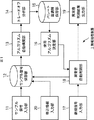•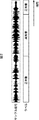•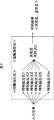•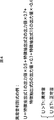•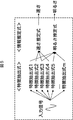••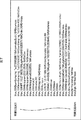•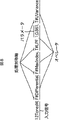•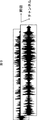•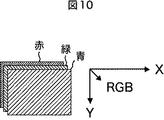••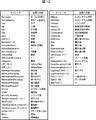•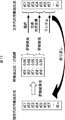•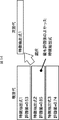•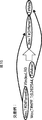•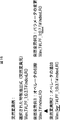•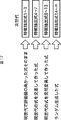•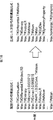•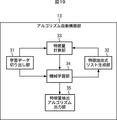•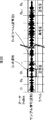•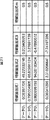•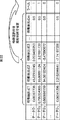•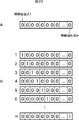•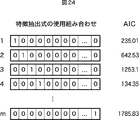•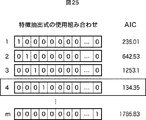•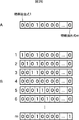••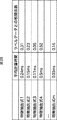•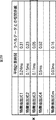•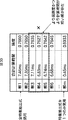•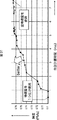•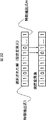•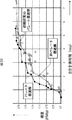•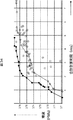•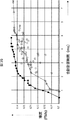•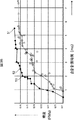•••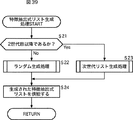•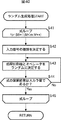•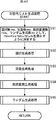•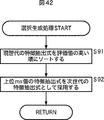•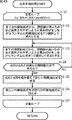•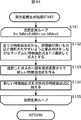•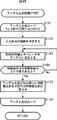•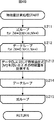•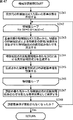•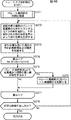•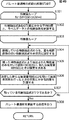•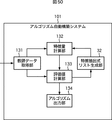•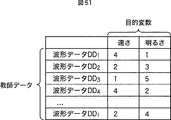•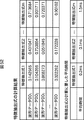••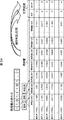•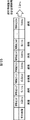•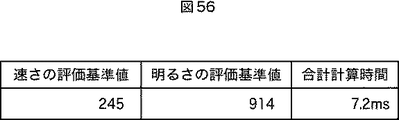•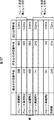•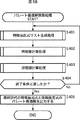•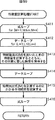•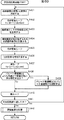•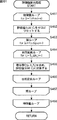•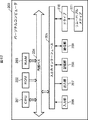## Classifications

• GPHYSICS
• G10MUSICAL INSTRUMENTS; ACOUSTICS
• G10LSPEECH ANALYSIS OR SYNTHESIS; SPEECH RECOGNITION; SPEECH OR VOICE PROCESSING; SPEECH OR AUDIO CODING OR DECODING
• G10L15/00Speech recognition
• G10L15/02Feature extraction for speech recognition; Selection of recognition unit

## Abstract

An information processing apparatus includes an algorithm configuring section that configures an algorithm for performing discrimination on an input signal by using a genetic search technique, and a tradeoff analyzing section that determines Pareto optimal solutions of the algorithm with respect to evaluation indices by performing tradeoff analysis on the basis of the algorithm.

## Description

The present invention relates to an information processing device, an information processing method, and a program, and in particular, an information processing device, an information processing method, and a program that can present a plurality of algorithms that have a trade-off relationship between a plurality of evaluation indexes. About.

Conventionally, a technique (Automatic Algorithm Generation Technology) for automatically constructing a feature quantity extraction algorithm for extracting a feature quantity representing a feature of given data by a genetic search method has been proposed. According to the algorithm automatic construction technique, algorithms for voice recognition, image recognition, and the like can be automatically constructed.

For example, the present applicant has proposed a technique for constructing an algorithm that can quickly extract a feature amount with high accuracy from content data such as music data (see, for example, Patent Document 1).

International Publication No. WO2007 / 049641

The conventional algorithm automatic construction technology outputs only the algorithm with the highest performance (highest accuracy). For example, when there are two evaluation indexes, performance (accuracy) and speed (processing time), the performance drops slightly. I couldn't get an algorithm that worked fast. Further, once the algorithm is constructed, the request for one evaluation index may be lowered, but it cannot cope with a change such as increasing the demand for the other evaluation index.

The present invention has been made in view of such a situation, and makes it possible to present a plurality of algorithms having a trade-off relationship between a plurality of evaluation indexes.

An information processing apparatus according to one aspect of the present invention includes a learning data acquisition unit that acquires sample signals and label data input as learning data, and at least one of selection generation, mutation generation, cross generation, and random generation A feature extraction formula generating means for generating a plurality of feature extraction formulas, which is a formula for performing a signal processing on the sample signal and extracting a predetermined feature amount using a genetic search method for performing the processing of: The feature quantity calculating means for extracting a feature quantity by processing according to the plurality of feature extraction formulas, and an information estimation formula for estimating the label data represented by a linear combination formula of the plurality of feature extraction formulas. Substituting the feature quantity obtained by the calculation means and the label data of the learning data to obtain a linear combination coefficient of the information estimation expression, and to the feature extraction expression generation means Among the plurality of generated feature extraction formulas, the label data is estimated using the processing time required for processing the feature extraction formulas to be used and the information estimation formula while changing the combination of the feature extraction formulas to be used. And a trade-off analysis means for obtaining a plurality of Pareto optimal solutions composed of the information estimation formula in which there is no highly evaluated solution for all evaluation indexes .

It is possible to further provide an optimum algorithm determining means for determining the information estimation formula comprising the optimal combination of the feature extraction formulas that match the requirements of the plurality of evaluation indexes from the plurality of Pareto optimal solutions.

The trade-off analysis unit generates an initial state solution of the Pareto optimal solution by deleting the feature extraction formulas one by one from the plurality of feature extraction formulas generated by the feature extraction formula generation unit , The Pareto optimal solution can be searched by the genetic search method using the initial state solution as an initial population .

The trade-off analysis means includes the genetic search using the plurality of feature extraction formulas generated by the feature extraction formula generation means as an initial state solution of the Pareto optimal solution and an initial population as the initial state solution. The Pareto optimal solution can be searched by the method .

In the trade-off analysis means, a Pareto optimal solution is obtained using at least the mutation generation that randomly changes the use or non-use of each feature extraction formula as the genetic search method using the solution of the initial state as an initial population. You can search .

Evaluation value obtaining Oite the information estimation equation, the contribution ratio is a ratio of the feature quantity extracted by the feature extraction equation contributed to estimate the label data of the learning data as an evaluation value of each feature extraction equation In the genetic search method, the feature extraction formula generation unit further includes a calculation unit so that a higher evaluation value of each feature extraction formula by the evaluation value calculation unit is more easily selected as an update target. The feature extraction formula constituting the information estimation formula can be updated.

The evaluation value calculation means includes a value obtained by summing up the contribution ratios of the feature extraction expressions in the plurality of Pareto optimal solutions obtained by the trade-off analysis means with respect to the same feature extraction expression. It can be made into evaluation value.

According to another embodiment of the present invention includes: a learning data obtaining unit, a feature extraction formula generation unit, a feature amount calculating means, and machine learning unit, the information processing method of an information processing apparatus and a trade-off analysis means The learning data acquisition unit acquires a sample signal and label data input as learning data, and the feature extraction formula generation unit includes at least one of selection generation, mutation generation, cross generation, and random generation. Generating a plurality of feature extraction formulas, which is a formula for performing signal processing on the sample signal and extracting a predetermined feature amount, using a genetic search method that performs two or more processes, and the feature amount calculation means, A feature amount is extracted by processing a sample signal according to the plurality of feature extraction formulas, and the machine learning means is expressed by a linear combination formula of the plurality of feature extraction formulas, and the label data Substituting the feature quantity obtained by the feature quantity calculation means and the label data of the learning data into an information estimation formula for estimating the characteristic estimation formula, obtaining a linear combination coefficient of the information estimation formula, and the trade-off analysis means, Among the plurality of feature extraction formulas generated by the extraction formula generation means, using the processing time required for processing the feature extraction formula to be used and the information estimation formula while changing the combination of the feature extraction formulas to be used The accuracy at the time of estimating the label data is used as an evaluation index, and a plurality of Pareto optimal solutions consisting of the information estimation formula for which there is no highly evaluated solution for all the evaluation indices are obtained .

A program according to one aspect of the present invention includes at least one of learning data acquisition means for acquiring a sample signal input as learning data and its label data, selection generation, mutation generation, cross generation, and random generation. Feature extraction formula generating means for generating a plurality of feature extraction formulas, which is a formula for performing signal processing on the sample signal and extracting a predetermined feature amount using a genetic search method for performing the above processing, and the sample signal The feature amount calculating means for extracting a feature amount by processing according to the plurality of feature extraction formulas, and the information estimation formula for estimating the label data represented by the linear combination formula of the plurality of feature extraction formulas. Machine learning means for substituting the feature data obtained by a quantity calculation means and label data of the learning data to obtain a linear combination coefficient of the information estimation formula; Among the plurality of feature extraction formulas generated by the output formula generation means, using the processing time required for processing the feature extraction formula to be used and the information estimation formula while changing the combination of the feature extraction formulas to be used The accuracy at the time of estimating the label data is used as an evaluation index, and it is made to function as a trade-off analysis means for obtaining a plurality of Pareto optimal solutions composed of the information estimation formulas that do not have a highly evaluated solution for all evaluation indexes .

In one aspect of the present invention, a genetic search method in which a sample signal input as learning data and its label data are acquired and at least one of selection generation, mutation generation, cross generation, and random generation is performed. , A plurality of feature extraction formulas are generated to perform signal processing on the sample signal and extract a predetermined feature quantity, and the feature quantity is extracted by processing the sample signal according to the plurality of feature extraction formulas, The linear combination coefficient of the information estimation formula, which is expressed by a linear combination formula of multiple feature extraction formulas, substituting the feature quantity obtained by the feature quantity calculation means and the label data of the learning data into the information estimation formula for estimating the label data Among the generated feature extraction formulas, the processing time required for processing the feature extraction formulas to be used and the information estimation are changed while changing the combination of the feature extraction formulas to be used. The accuracy at the time of estimating label data using a formula is used as an evaluation index, and a plurality of Pareto optimal solutions consisting of the information estimation formula for which there is no highly evaluated solution for all evaluation indices are obtained.

According to one aspect of the present invention, it is possible to present a plurality of algorithms that have a trade-off relationship between a plurality of evaluation indexes.

FIG. 1 shows a configuration example of an embodiment of an information processing apparatus to which the present invention is applied.

The information processing apparatus 1 is a device that performs processing for detecting abnormal sound, automatically constructs an algorithm based on a sample signal with known abnormal sound and normal sound input as learning data, and follows the automatically constructed algorithm. Then, it is determined whether a new input signal (hereinafter referred to as a new signal) is an abnormal sound or a normal sound.

The information processing apparatus 1 includes a sample signal input unit 11, a sample signal storage unit 12, an algorithm automatic construction unit 13, a trade-off analysis unit 14, a Pareto optimal solution storage unit 15, a use algorithm determination unit 16, a new signal input unit 17, an abnormality The automatic sound determination unit 18, the abnormal sound determination result output unit 19, and the correction information input unit 20 are configured.

The sample signal input unit 11 receives a sample signal for algorithm construction input from another block (not shown) of the information processing apparatus 1 or another device, and supplies it to the sample signal storage unit 12.

The sample signal storage unit 12 stores a sample signal as teacher data supplied from the sample signal input unit 11. Signals supplied from the abnormal sound automatic determination unit 18 and the correction information input unit 20 to the sample signal storage unit 12 will be described later.

Data representing whether the signal is a normal sound or an abnormal sound is added to the sample signal input to the sample signal input unit 11 as a label.

FIG. 2 shows an example of the sample signal input to the sample signal input unit 11. The horizontal axis in FIG. 2 represents time, and the sample signal and the label have a one-to-one correspondence at each time. Note that a label indicating whether the sound is a normal sound or an abnormal sound can be given as a signal of a high level or a low level, for example.

The algorithm automatic construction unit 13 performs learning based on a genetic search method to determine whether an abnormal sound or a normal sound is an abnormal sound or a normal sound with respect to a new signal that is unknown as to whether it is an abnormal sound or a normal sound. Build (generate) an algorithm to discriminate. Details of the algorithm construction will be described later with reference to FIG. 3 and the like. The constructed algorithm is an algorithm (feature quantity extraction algorithm) that extracts a feature quantity representing a predetermined feature of the input signal, and is extracted by the algorithm. An abnormal sound or a normal sound can be discriminated based on whether or not the feature value (value) is equal to or greater than a predetermined threshold value.

The trade-off analysis unit 14 performs a trade-off analysis based on the algorithm constructed by the algorithm automatic construction unit 13 to obtain a Pareto optimal solution. Then, the trade-off analysis unit 14 causes the Pareto optimal solution storage unit 15 to store a plurality of algorithms as Pareto optimal solutions. The Pareto optimal solution storage unit 15 stores a plurality of algorithms as Pareto optimal solutions supplied from the trade-off analysis unit 14.

The use algorithm determination unit 16 includes a plurality of evaluation index requirement conditions such as the current free resource status of the information processing apparatus 1 and the required performance among the plurality of algorithms stored in the Pareto optimal solution storage unit 15. An optimal algorithm that matches the above is determined and supplied to the abnormal sound automatic discrimination unit 18.

The new signal input unit 17 receives an input of a new signal that is a target for detecting whether or not the sound is abnormal, and supplies the input to the abnormal sound automatic determination unit 18.

The abnormal sound automatic determination unit 18 determines whether the new signal supplied from the new signal input unit 17 is an abnormal sound or a normal sound using the algorithm supplied from the use algorithm determination unit 16. The discrimination result is supplied to the abnormal sound discrimination result output unit 19. Further, the abnormal sound automatic discrimination unit 18 adds the discrimination result as a label to the new signal supplied from the new signal input unit 17 and supplies the new signal to the sample signal storage unit 12 for storage. That is, the new signal supplied from the new signal input unit 17 is stored in the sample signal storage unit 12 as a new sample signal.

The abnormal sound determination result output unit 19 outputs the determination result supplied from the abnormal sound automatic determination unit 18 as audio or video. For example, the abnormal sound discrimination result output unit 19 outputs a sound indicating that the new signal is an abnormal sound when the new signal is an abnormal sound, or displays a video image displaying characters of “normal sound” or “abnormal sound”. To display.

When there is an error in the label of the sample signal stored in the sample signal storage unit 12, the correction information input unit 20 corrects the label. That is, the correction information input unit 20 receives a user operation and rewrites the label of the sample signal.

Therefore, in addition to the labeled sample signal supplied from the sample signal input unit 11, the sample signal storage unit 12 is also supplied with the labeled sample signal from the abnormal sound automatic determination unit 18, and the abnormal sound automatic determination unit 18. If there is an error in the label of the sample signal supplied from, the correction information input unit 20 corrects the label.

Next, algorithm construction performed by the algorithm automatic construction unit 13 will be described in detail.

FIG. 3 is a diagram for explaining the structure of the constructed algorithm.

The constructed algorithm is composed of m feature extraction formulas 1 to m (m is an integer greater than 1) and an information estimation formula expressed by a linear combination formula of the m feature extraction formulas 1 to m. The The feature extraction formula performs a predetermined calculation on the input signal and outputs a one-dimensional value as the calculation result. The information estimation formula estimates information indicating the feature of the input signal using a one-dimensional value that is an output value of the feature extraction formula. The algorithm automatic construction unit 13 obtains an abnormal sound discriminant U that is an information estimation formula.

FIG. 4 shows a specific example of the abnormal sound discriminant U. Since the determination result to be output is a binary value indicating whether it is an abnormal sound or a normal sound, the calculation result of the abnormal sound discriminant U is equal to or less than a predetermined threshold Th, or a predetermined value Whether the input signal is a normal sound or an abnormal sound is determined depending on whether it is larger than the threshold value Th. For example, as shown in FIG. 4, when the calculation result of the abnormal sound discriminant U is equal to or less than a predetermined threshold Th, a determination result that the input signal is a normal sound is output, and the predetermined threshold Th is used. If it is larger, a determination result that the input signal is an abnormal sound is output. In the example of the abnormal sound discriminant U shown in FIG. 4, among the feature extraction formulas 1 to m, only the feature extraction formulas 1, 2, 5, and 15 are used. This is because the linear combination coefficient of the equation is zero.

It should be noted that the constructed algorithm is not a binary value such as whether it is an abnormal sound or a normal sound, but is within a predetermined range such as the speed of music or the level of brightness (level). Some estimate the value of. In this case, as shown in FIG. 5, the value representing the speed of the input signal is obtained from the calculation result of the speed estimation formula represented by the linear combination formula of m feature extraction formulas 1 to m. Is obtained from the calculation result of the brightness estimation formula expressed by a linear combination of m feature extraction formulas 1 to m.

In the next embodiment to be described later with reference to FIG. 50 and thereafter, as shown in FIG. 6, when a new signal is input, an algorithm used as a feature extractor for extracting the speed and brightness values. An example of constructing will be described.

FIG. 7 shows examples of the feature extraction formulas 1 to m.

The feature extraction expression is an expression that can process an input signal that can be expressed in a plurality of dimensions until it reaches a single value, that is, a scalar quantity. FIG. 8 shows an example of the feature extraction formula.

The feature extraction formula is configured by an operator which is information indicating the type of input signal and information indicating signal processing to be performed on the input signal. The parameters in are included. In the example of FIG. 8, 12TonesM is information indicating the type of input data, and the following four F # Differential, F # MaxIndex, T # LPF_1; 0.861, and T # UVariance are operators.

As information indicating the type of the input signal, in addition to 12TonesM, which represents a pitch analysis of the monaural PCM (pulse coded modulation sound source) waveform data shown in the example of FIG. There are Wav for representing, Spectrum for representing a two-dimensional image that is a spectrum of sound, and the like.

The feature extraction formula indicates that the signal processing represented by the operator is sequentially performed on the input signal from the side closer to the information indicating the type of the input signal. A processing target axis (target processing axis) is described at the head of the operator. The axis to be processed represents that T is in the time direction and F is the frequency (pitch direction).

The processing contents following the operator's symmetry axis are: Differential represents differentiation, MaxIndex represents the maximum value index, LPF_1 represents low-pass filtering, and UVariance computes unbiased variance. Represents. Each operator is given parameters as necessary. In the example of FIG. 8, a parameter of 0.861 is assigned to LPF_1 (low-pass filtering) processing.

Therefore, the feature extraction formula of FIG. 8 differentiates the data obtained by analyzing the pitch of the PCM waveform data along the time axis in the pitch direction, and calculates an index indicating the maximum value in the pitch direction (the pitch of the strongest pitch). Obtain a number), apply a low-pass filter in the time axis direction, calculate the unbiased variance at the end, and output the calculation result.

In this embodiment, the input signal employs Wav representing a waveform with a signal representing sound, but the feature extraction formula is not only a sound signal but also a matrix format (a value is contained in a multidimensional matrix). Any image can be used as long as it can be expressed in a format).

For example, the input signal is an audio waveform signal having a matrix of amplitude, time, and channel as shown in FIG. 9, and the X-axis, Y-axis, RGB (R: red) of the frame as shown in FIG. , G: green, B: blue) image signal captured as a matrix, and as illustrated in FIG. 11, a video signal captured as a frame X-axis, Y-axis, RGB, and time matrix. It is also applicable to cases. Furthermore, binary data that can be expressed in a matrix may be used.

Note that the types of operators include those shown in FIG. For example, the operator types include mean value (Mean), fast Fourier transform (FFT), standard deviation (StDev), appearance rate (Ratio), low-pass filter (LPF_1), high-pass filter (HPF_1), absolute value (ABS), There are square (Sqr), square root (Sqrt), normalization (Normalize), differentiation (Differential), integration (Integrate), maximum value (MaxIndex), unbiased variance (UVariance), downsampling (DownSampling), and the like. Depending on the determined operator, the process symmetry axis may be fixed, and in this case, the process symmetry axis fixed to the operator is adopted. When an operator who needs a parameter is determined, the parameter is also determined to be a random value or a preset value.

Next, with reference to FIG. 13 to FIG. 18, the flow of automatic algorithm generation performed by the automatic algorithm construction unit 13 based on the genetic search method will be described.

As shown in FIG. 13, the algorithm automatic construction unit 13 calculates an evaluation value for evaluating each feature extraction formula for the feature extraction formulas 1 to m of the current generation. Then, the algorithm automatic construction unit 13 rearranges the current generation feature extraction formulas 1 to m in descending order of evaluation values, and then performs selection generation, cross generation, mutation generation, and random generation to generate the next generation. Feature extraction formulas 1 to m are generated. The reason why the current generation feature extraction formulas 1 to m are rearranged in descending order of the evaluation value is to select the feature extraction formula for generating the next generation feature extraction formula from the one with the highest evaluation value. is there. Then, the next generation feature extraction formulas 1 to m become the current generation feature extraction formulas 1 to m, and the generation of the next generation feature extraction formulas 1 to m based on the current generation feature extraction formulas 1 to m is repeated.

Selection generation, cross generation, mutation generation, and random generation will be described in detail.

Select generation is to generate a new feature extraction formula by selecting a predetermined number of feature extraction formulas in order from the highest evaluation value among the current generation feature extraction formulas as the next generation feature extraction formulas. Represents.

For example, as shown in FIG. 14, when the evaluation values of the current generation feature extraction formulas 1, 2, and 3 are 0.53, 0.85, and 0.74, respectively, the current generation having the highest evaluation value Feature extraction formula 2 is selected as the next-generation feature extraction formula 1.

Intersection generation is performed by rearranging (intersecting) two feature extraction formulas randomly selected by weighting so that the higher evaluation value of the current generation feature extraction formulas can be selected. Represents generating a new feature extraction formula.

For example, as shown in FIG. 15, the first feature extraction formula “Wav, T # Differential, T # IndexLR0” and the second feature extraction formula “Wav, T # HPF_1; 0.262544, T #” having a high evaluation value are used. Mean ”is selected, and the“ Wav, T # Differential ”part of the first feature extraction formula and the“ T # Mean ”part of the second feature extraction formula are combined, in other words, the first feature extraction formula The “T # IndexLR0” part is rearranged with the “T # Mean” part of the second feature extraction formula to generate a new feature extraction formula.

Mutation generation is a new feature by randomly changing a part of the feature extraction formula selected at random by weighting so that the higher evaluation value of the current generation feature extraction formula can be selected. Indicates that an extraction formula is generated.

For example, as shown in FIG. 16, a feature extraction formula “Wav, T # LPF_1; 0.3, T # IndexLR0” having a high evaluation value is selected as a mutation source, and one operator from the feature extraction formula is selected. “T # LPF_1; 0.3” is deleted (mutation example 1), operator “Sqr” is added (mutation example 2), and one operator “T # LPF_1; 0.3” in the feature extraction formula The parameter is changed to 0.7 (mutation example 3), and a new feature extraction formula is generated.

Random generation indicates that a new feature extraction formula is generated by combining operators randomly so that the input signal becomes one value (scalar amount). Note that the first generation feature extraction formulas 1 to m are all generated by random generation since there is no previous generation feature extraction formula.

Among the above selection generation, intersection generation, mutation generation, and random generation, for example, the algorithm automatic construction unit 13 obtains the next generation feature extraction formulas 1 to 3 by selection generation as shown in FIG. The next generation feature extraction formulas 4 to 7 are obtained by cross generation, the next generation feature extraction formulas 8 to 13 are obtained by mutation generation, and the next generation feature extraction formulas 1 to m are obtained by random generation.

FIG. 18 shows an example in which the next generation feature extraction formulas 1 to m are generated from the current generation feature extraction formulas 1 to m.

FIG. 19 is a block diagram illustrating a detailed configuration example of the algorithm automatic construction unit 13.

The algorithm automatic construction unit 13 includes a learning data cutout unit 31, a feature extraction formula list generation unit 32, a feature amount calculation unit 33, a machine learning unit 34, and a feature amount extraction algorithm output unit 35.

The learning data cutout unit 31 cuts out the learning data stored in the sample signal storage unit 12 and supplies it to the feature amount calculation unit 33 and the machine learning unit 34. That is, the learning data cutout unit 31 divides the sample signal stored in the sample signal storage unit 12 and the label thereof every predetermined unit time, and sends the divided sample signal of each unit time to the feature amount calculation unit 33. Then, the label of each unit time is supplied to the machine learning unit 34.

Now, as shown in FIG. 20, it is assumed that the sample signal stored in the sample signal storage unit 12 and its label are divided into n unit times by the learning data cutout unit 31. the sample signal of each unit time, respectively, referred to as data D 1 through data D n, a label for each data D 1 through data D n is referred to as the label data. In this case, learning data extraction unit 31 supplies the data D 1 through data D n in the feature amount calculating unit 33 supplies the label data of the data D 1 through data D n to the machine learning unit 34.

The feature extraction formula list generation unit 32 generates the next generation feature extraction formulas 1 to m based on the evaluation values of the current generation feature extraction formulas 1 to m supplied from the machine learning unit 34, and the feature amount calculation unit 33. Specifically, the feature extraction formula list generation unit 32 rearranges the current generation feature extraction formulas 1 to m in descending order of evaluation values, and performs selection generation, cross generation, and sudden generation for a predetermined feature extraction formula of the current generation. Next generation feature extraction formulas 1 to m are generated by performing mutation generation and random generation.

The feature amount calculation unit 33 calculates the feature extraction formulas 1 to m supplied from the feature extraction formula list generation unit 32 for each of the data D 1 to data D n supplied from the learning data cutout unit 31, the calculation result is supplied to the machine learning unit 34 as the feature amount data D 1 through data D n.

FIG. 21 shows an example of the feature amount calculated by the feature amount calculation unit 33 and supplied to the machine learning unit 34.

The machine learning unit 34 uses each feature amount of the data D 1 to data D n supplied from the feature amount calculation unit 33 and the label data of the data D 1 to data D n supplied from the learning data cutout unit 31. Thus, the abnormal sound discriminant U is estimated by linear discrimination with feature selection. In other words, by using the characteristic amounts of the supplied data D 1 through data D n from the feature quantity calculating unit 33, best fits the label data of the supplied data D 1 through data D n from the learning data extraction unit 31 The abnormal sound discriminant U using the feature extraction formula is estimated. Further, the machine learning unit 34 calculates an evaluation value of each feature extraction formula when the estimated abnormal sound discriminant U is used, and supplies the evaluation value to the feature extraction formula list generation unit 32.

The process of the machine learning unit 34 will be described in more detail.

FIG. 22 illustrates an example of data supplied from the feature amount calculation unit 33 and the learning data cutout unit 31 to the machine learning unit 34.

Machine learning unit 34 of the feature extraction expressions 1 to m of the current generation, and evaluate the case where any feature extraction was not the case or using using equation best fit the label data of the data D 1 through data D n, Determine the optimal combination of feature extraction formulas to use.

First, as shown in FIG. 23A, the machine learning unit 34 uses a state in which all of the current generation feature extraction formulas 1 to m are not used (a state in which the feature extraction formulas 1 to m are not used) as a reference state. Based on the state, use combinations of m feature extraction formulas, which are shown in FIG. 23B, in which use / unuse of each feature extraction formula are inverted one by one, are determined. In FIG. 23A and FIG. 23B, the arrangement of 0 or 1 in one row is represented by “1” when each feature extraction formula of the feature extraction formulas 1 to m is used, and “0” when not used. Yes, in order from the left side of one row, the feature extraction formulas 1, 2, 3,..., M are used or not used.

Next, the machine learning unit 34 generates (estimates) an abnormal sound discriminant U for each use combination of m feature extraction formulas. The abnormal sound discriminant U is expressed by the following equation (1), where X 1 to X m are feature amounts based on the feature extraction equations 1 to m , b 1 to b m are linear combination coefficients, and b 0 is an intercept. The

U = b 1 X 1 + b 2 X 2 + b 3 X 3 +... + B m X m + b 0 (1)

In equation (1), the value of the linear combination coefficient for the unused feature quantity is zero.

In order to obtain the linear combination coefficients b 1 to b m and the intercept b 0 , the least square method can be used. That is, the k-th label data (true value) of the label data of the data D 1 to data D n supplied from the feature amount calculation unit 33 is expressed as U k and the true value obtained by Expression (1). ', the prediction error e k is e k = {U k -U k ' the predictive value of U k U k can be expressed by}. Then, by obtaining the linear combination coefficients b 1 to b m and the intercept b 0 square error E = Σe k 2 is the minimum prediction error e k for each label data of the data D 1 through data D n, optimum The linear combination coefficients b 1 to b m and the intercept b 0 can be obtained.

The obtained abnormal sound discriminant U is, for example, the following formula.

U = 0.5 × X 1 + 0.3 × X 2 + 0.0 × X 3 +... + 0.7X m +0.2
In the example of the abnormal sound discriminant U, the linear coupling coefficients b 1 , b 2 , b 3 ,..., B m , b 0 are 0.5, 0.3, 0.0,. , 0.7, 0.2, and the feature quantity based on the feature extraction formula 3 having a linear combination coefficient of zero is unused.

Next, the machine learning unit 34 calculates an evaluation value of the abnormal sound discriminant U generated for each use combination of the m feature extraction formulas. Here, the evaluation value of the abnormal sound discriminant U is referred to as an evaluation reference value in order to distinguish it from the evaluation value of each feature extraction formula.

For example, AIC (Akaike Information Criteria) can be adopted as the evaluation function for obtaining the evaluation reference value. AIC is a function indicating that a smaller value is better (higher evaluation), and is calculated by the following equation (2).

In Expression (2), n represents the number of teacher data, that is, the number of data supplied from the learning data cutout unit 31, and PI represents the circumference ratio. Further, ( || E || 2 / n) represents a mean square error with respect to each data of data D 1 to data D n , and m ′ represents the number of feature extraction formulas in use.

FIG. 24 shows the evaluation reference value of each abnormal sound discriminant U calculated for each use combination of the m feature extraction formulas of FIG. 23B.

The machine learning unit 34 selects the use combination of the feature extraction formula having the highest evaluation (the evaluation standard value is the smallest) as the next reference state. In the example shown in FIG. 24, as shown in FIG. 25, since the evaluation reference value of the abnormal sound discriminant U based on the use combination in which only the feature extraction formula 4 is used is the smallest, the machine learning unit 34 The use combination for which only the feature extraction formula 4 is used is selected as the next reference state.

Then, the machine learning unit 34 determines use / unuse of each feature extraction formula shown in FIG. 26B for the use combination in which only the feature extraction formula 4 that is the reference state shown in FIG. 26A is used. Use combinations of m feature extraction formulas that are inverted one by one are determined.

The machine learning unit 34 generates (estimates) an abnormal sound discriminant U for each of the determined combinations of m feature extraction formulas, calculates an evaluation reference value thereof, and determines the next reference state repeat. The determination of the reference state, generation of the abnormal sound discriminant U, and calculation of the evaluation reference value are repeated until the evaluation reference value is not updated, that is, until a smaller evaluation reference value is not calculated.

When the abnormal sound discriminant U whose evaluation reference value is no longer updated is obtained, the machine learning unit 34 evaluates each of the feature extraction formulas 1 to m based on the linear combination coefficient of the obtained abnormal sound discriminant U. Calculate the value.

Specifically, the machine learning unit 34 calculates the contribution rate C i of the i-th feature amount represented by Expression (3) as an evaluation value of the feature extraction expression i.

C i = b i ÷ StDev (X i ) × StDev (T) × Correl (X i , T) (3)

Here, StDev (X i ) represents the standard deviation of the value (feature quantity X i ) of the feature extraction formula i in the data D 1 to data D n , and StDev (T) represents the data D 1 to data D n . The standard deviation of the label data T is represented. Correl (T) represents the value of the feature extraction formula i (feature amount X i ) in the data D 1 to data D n and the Pearson correlation coefficient of the label data T.

The evaluation values of the feature extraction formulas 1 to m calculated as described above are supplied to the feature extraction formula list generation unit 32 together with the generated abnormal sound discriminant U.

Further, when a predetermined end condition is reached, for example, when a process for generating a next-generation feature extraction formula is performed a predetermined number of times, the machine learning unit 34 uses the feature generation formula for the final generation and the abnormal sound discrimination formula U as a feature amount. This is supplied to the extraction algorithm output unit 35. The feature extraction formula of the final generation is acquired from the feature extraction formula list generation unit 32 or the feature amount calculation unit 33.

The feature quantity extraction algorithm output unit 35 supplies the final generation feature extraction formula and the abnormal sound discriminant U supplied from the machine learning unit 34 to the trade-off analysis unit 14 (FIG. 1).

Next, detailed processing of the trade-off analysis unit 14 will be described.

The trade-off analysis unit 14 uses the algorithm (abnormal sound discriminant U) constructed by the algorithm automatic construction unit 13 as the first one Pareto optimal solution, and trades off using two evaluation indexes of algorithm execution speed and accuracy. Through analysis, a new Pareto optimal solution is obtained. In the search for the Pareto optimal solution when a new Pareto optimal solution is obtained, the trade-off analysis unit 14 generates the next Pareto optimal solution candidate only by mutation from the feature amount extraction algorithm as the current Pareto optimal solution. Adopt a simple genetic algorithm.

The Pareto optimal solution will be described with reference to FIG.

When an algorithm is evaluated using two evaluation indexes, if there is no other highly evaluated solution for all evaluation indexes, it can be said that the solution is a Pareto optimal solution. On the other hand, when there are other highly evaluated solutions for all evaluation indexes, the solutions are not Pareto optimal solutions. In FIG. 27, the Pareto optimal solution is indicated by a black circle, and the non-Pareto optimal solution is indicated by a white circle.

In order to obtain a plurality of abnormal sound discriminants U as Pareto optimal solutions as shown in FIG. 27, the trade-off analysis unit 14 first initializes the Pareto optimal solutions. That is, the trade-off analysis unit 14 is a feature extraction formula having a low correlation with the label data (the above-mentioned Correl (T)) among the feature extraction formulas included in the abnormal sound discriminant U constructed by the algorithm automatic construction unit 13. Are set to unused one by one in order, and the maximum number of feature extraction formulas included in the abnormal sound discriminant U constructed by the algorithm automatic construction unit 13 is used from the maximum number to one. A plurality of abnormal sound discriminants U each having a different number of feature extraction formulas are generated.

Generating a plurality of abnormal sound discriminants U with different numbers of feature extraction formulas to be used one by one based on the abnormal sound discriminant U constructed by the automatic algorithm construction unit 13 is called initialization of the Pareto optimal solution. Since the abnormal sound discriminant U supplied from the algorithm automatic construction unit 13 does not always use all the feature extraction formulas, the feature extraction included in the abnormal sound discriminant U constructed by the algorithm automatic construction unit 13 is performed. Although the number of expressions may be smaller than m, in the present embodiment, an abnormal sound discriminant U using all feature extraction expressions is supplied from the algorithm automatic construction unit 13 in order to simplify the explanation. It will be described as being done.

The initialization of the Pareto optimal solution will be further described with reference to FIGS.

As shown in FIG. 28, the trade-off analysis unit 14 displays a list of correlation coefficients between the average time (average calculation time) required for calculating each feature extraction formula and the label data calculated by each feature extraction formula. create. The average calculation time can be obtained by inputting data D 1 to data D n and calculating, and the correlation coefficient can be obtained by Correl (T) in Expression (3).

Next, the trade-off analysis unit 14 sets the feature extraction formula having the lowest correlation coefficient among the used feature extraction formulas to be unused, and uses only the feature quantities extracted by the remaining feature extraction formulas. Thus, the linear combination coefficients b 1 to b m and the intercept b 0 are obtained, and a new abnormal sound discriminant U is determined. Further, the rate-off analysis unit 14 also calculates time (total calculation time) and accuracy (estimated accuracy) required for discrimination when abnormal sound discrimination is performed using the determined new abnormal sound discriminant U.

In the example of data shown in FIG. 28, since the correlation coefficient of the feature extraction formula 3 is “0.05”, which is the lowest, the feature extraction formula 3 is set to unused as shown in FIG. Then, a new abnormal sound discriminant U and its accuracy are determined when using the feature extraction formulas 1 to m excluding the feature extraction formula 3, and the total calculation excluding the calculation time (0.11 ms) required for the feature extraction formula 3 Time is calculated.

Similarly, the feature extraction formulas are set to unused one by one in descending order of correlation coefficient, a new abnormal sound discriminant U, the total calculation time and accuracy are calculated, and the Pareto optimal solution candidates in FIG. Is created.

The trade-off analysis unit 14 extracts only the Pareto optimal solution from the list of Pareto optimal solution candidates shown in FIG. 30 (deletes the non-Pareto optimal solution). Specifically, the abnormal sound discriminant U from solution 1 using m feature extraction formulas to solution m using only one feature extraction formula, as candidates for Pareto optimal solutions, shown in FIG. Among them, solutions 3 to 5 having other solutions with higher accuracy and a short total calculation time are deleted as non-Pareto optimal solutions, and the remaining solutions are set as Pareto optimal solutions.

In general, as the number of feature extraction formulas decreases, the total calculation time decreases but the accuracy tends to decrease, so the sum of multiple abnormal sound discriminants U generated by initialization of the Pareto optimal solution When the calculation time and accuracy are graphed, it is as shown in FIG. The horizontal axis of the graph of FIG. 31 is the total calculation time (ms), and the vertical axis is the accuracy based on the F value (FMs: F-Measures).

By initializing the Pareto optimal solution, the Pareto optimal solution in the initial state is determined.

Next, the trade-off analysis unit 14 generates a new solution by mutating a plurality of Pareto optimal solutions as an initial state, and updates the Pareto optimal solution to search for a better Pareto optimal solution. When updated to a better Pareto optimal solution, the line connected by the Pareto optimal solution moves in the upper left direction as indicated by an arrow in FIG.

Specifically, the trade-off analysis unit 14 randomly selects one solution from among a plurality of Pareto optimal solutions as an initial state, and a plurality of the selected solutions (selected solutions) (for example, 1 to 1). For the (3) feature extraction formulas, a new solution is generated in which used or unused is randomly changed.

FIG. 32 shows an example of a mutation in which a new solution is generated by changing the feature extraction formula 7 of the selected solution from use to unused and changing the feature extraction formula 8 from unused to use.

Then, the trade-off analysis unit 14 calculates the total calculation time and accuracy for the new solution, re-creates the list of FIG. 30, and deletes the non-Pareto optimal solution.

The trade-off analysis unit 14 selects one solution again at random for the remaining Pareto optimal solution, generates a new solution by mutation based on the selected solution, and searches for a better Pareto optimal solution. The process is executed until the end condition is satisfied, for example, until a predetermined number of repetitions is reached.

FIG. 33 shows the Pareto optimal solution after the process of searching for the Pareto optimal solution is repeatedly executed 200 times from the Pareto optimal solution in the initial state.

In FIG. 33, the solid line connecting the black circles is the Pareto optimal solution after repeated execution 200 times, and the dotted line indicates the Pareto optimal solution in the initial state. A white circle (◯) indicates a non-Pareto optimal solution calculated in the search process.

FIG. 34 similarly shows the Pareto optimal solution after repeatedly executing the process of searching for the Pareto optimal solution 1000 times, and FIG. 35 shows the Pareto optimal solution after repeatedly executing 5000 times. As the number of iterations is increased, a better Pareto optimal solution is obtained. However, a large difference is not observed after 1000 executions and 5000 executions, and it can be seen that a sufficient Pareto optimal solution can be obtained after a predetermined number of executions.

Next, processing of the use algorithm determination unit 16 and the abnormal sound automatic determination unit 18 will be described.

The use algorithm determination unit 16 is obtained by the trade-off analysis unit 14 as described above, and from among a plurality of algorithms (abnormal sound discriminant U) as Pareto optimal solutions stored in the Pareto optimal solution storage unit 15, Based on the required processing time and accuracy, an optimal algorithm is determined and supplied to the abnormal sound automatic discrimination unit 18.

For example, as shown in FIG. 36, when the resource has a margin and the highest performance (maximum accuracy) is required even if processing time is required, the use algorithm determination unit 16 determines the Pareto optimal solution with the highest accuracy. 51 algorithms (abnormal sound discriminant U) are determined and supplied to the abnormal sound automatic discrimination unit 18.

Further, for example, when the use algorithm determining unit 16 is required to have an accuracy of 0.76 (FMs) or more, the Pareto optimal with the shortest total calculation time (processing time) is satisfied while satisfying the request. The algorithm of the solution 52 (abnormal sound discriminant U) is determined and supplied to the abnormal sound automatic discrimination unit 18.

Further, when the processing time per data is required to be 2 (ms) or less as the total calculation time (processing time), the use algorithm determining unit 16 determines the algorithm (abnormal sound) of the Pareto optimal solution 53. A discriminant U) is determined and supplied to the abnormal sound automatic discrimination unit 18.

As shown in FIG. 37, the abnormal sound automatic determination unit 18 divides the new signal supplied from the new signal input unit 17 into unit times similar to the learning data cutout unit 31, and each divided unit time The new signal is discriminated whether it is an abnormal sound or a normal sound by using the algorithm supplied from the use algorithm determining unit 16, and the discrimination result is supplied to the abnormal sound discrimination result output unit 19. As described above, the determination result for each unit time of the new signal is also supplied to and stored in the sample signal storage unit 12.

Next, the algorithm automatic construction process performed by the algorithm automatic construction unit 13 will be described with reference to the flowchart of FIG.

First, in step S <b> 1, the learning data cutout unit 31 cuts out the learning data stored in the sample signal storage unit 12 and supplies it to the feature amount calculation unit 33 and the machine learning unit 34. Specifically, the learning data extraction unit 31, the data D 1 through data D n cut from the learning data supplied to the feature quantity calculation unit 33, the label data of the data D 1 through data D n to the machine learning unit 34 Supply.

In step S2, the feature extraction formula list generation unit 32 generates the next generation feature extraction formulas 1 to m (feature extraction formulas) based on the evaluation values of the current generation feature extraction formulas 1 to m supplied from the machine learning unit 34. A feature extraction formula list generation process for generating (list) is executed. Of the repeated processing in step S2, the next generation feature extraction formulas 1 to m are generated by random generation in the first step S2 processing in which the current generation feature extraction formulas 1 to m do not exist. Detailed processing of the feature extraction formula list generation processing will be described later with reference to FIG.

In step S <b> 3, the feature amount calculation unit 33 executes a feature amount calculation process. That is, the feature amount calculation unit 33 calculates the data D 1 to data D n supplied from the learning data cutout unit 31 according to the feature extraction formulas 1 to m supplied from the feature extraction formula list generation unit 32. the results, supplies the machine learning unit 34 as the feature amount data D 1 through data D n. Detailed processing of the feature amount calculation processing will be described later with reference to FIG.

In step S4, the machine learning unit 34 executes a machine learning process. That is, the machine learning unit 34 uses the feature amounts of the data D 1 to data D n supplied from the feature amount calculation unit 33 to label the data D 1 to data D n supplied from the learning data cutout unit 31. The abnormal sound discriminant U using the feature extraction formula that best fits the data is estimated. Further, the machine learning unit 34 calculates an evaluation value of each feature extraction formula when the estimated abnormal sound discriminant U is used, and supplies the evaluation value to the feature extraction formula list generation unit 32. Detailed processing of the machine learning processing will be described later with reference to FIG.

In step S5, the machine learning unit 34 determines whether an end condition has been reached. Specifically, for example, when the processes in steps S2 to S4 described above are repeatedly executed a predetermined number of times or an operation to end is detected, the machine learning unit 34 determines that the end condition has been reached.

If it is determined in step S5 that the end condition has not been reached, the process returns to step S2, and the subsequent processes are repeated.

On the other hand, if it is determined in step S5 that the end condition has been reached, the process proceeds to step S6, and the machine learning unit 34 uses the feature extraction algorithm output unit 35 to extract the feature extraction formula and the abnormal sound discriminant U of the last generation. To supply. The feature quantity extraction algorithm output unit 35 outputs the final generation feature extraction formula and the abnormal sound discriminant U to the trade-off analysis unit 14 and ends the processing.

Next, the feature extraction formula list generation process executed in step S2 of FIG. 38 will be described with reference to the flowchart of FIG.

In step S <b> 21, the feature extraction formula list generation unit 32 determines whether the generated feature extraction formula list is the second generation or later.

If it is determined in step S21 that it is not the second generation or later, that is, the first generation, the process proceeds to step S22, and the feature extraction formula list generation unit 32 performs random generation, which will be described later with reference to FIG. Execute the process.

If it is determined in step S21 that the generation is the second or later generation, the process proceeds to step S23, and the feature extraction formula list generation unit 32 executes a next generation list generation process to be described later with reference to FIG.

After step S22 or step S23, the feature extraction formula list generator 32 supplies the feature extraction formula list generated by the process of step S22 or step S23 to the feature quantity calculator 33 in step S24. Returns to step S2 of FIG. 38 and proceeds to step S3.

As described above, in the first generation, all feature extraction formulas are randomly generated, and in the second and subsequent generations, a next-generation list generation process using a genetic search method is executed.

Next, the random generation process executed in step S22 in FIG. 39 will be described with reference to the flowchart in FIG.

In step S41, the feature extraction formula list generation unit 32 initializes the formula loop parameter M to 1 and starts the formula loop. The formula loop is repeated for the number m of feature extraction formulas constituting the feature extraction formula list.

In step S42, the feature extraction formula list generation unit 32 determines the type of the input signal of the Mth feature extraction formula (hereinafter also referred to as feature extraction formula [M]). In the present embodiment, the type of input signal is determined as Wav representing a waveform.

In step S43, the feature extraction formula list generation unit 32 randomly determines one processing symmetry axis and one operator of the feature extraction formula [M] to be generated.

In step S44, the feature extraction formula list generation unit 32 determines whether the calculation result of the feature extraction formula [M] generated so far is a scalar quantity (one-dimensional). If it is determined in step S44 that the calculation result is not a scalar quantity, the process returns to step S43, the subsequent processes are repeated, and one operator is added.

If it is determined in step S44 that the calculation result is a scalar quantity, the feature extraction formula list generation unit 32 determines in step S45 whether the formula loop parameter M is smaller than the maximum value m. If it is determined in step S45 that the expression loop parameter M is smaller than the maximum value m, the expression loop parameter M is incremented by 1, the process returns to step S42, and the subsequent processes are repeated.

On the other hand, if it is determined in step S45 that the expression loop parameter M is not smaller than the maximum value m (the expression loop parameter M is the same value as the maximum value m), the process exits the expression loop and the processing is performed in the step of FIG. It returns to S22 and progresses to step S24.

Through the above processing, a first generation feature extraction formula list is generated.

Next, the next generation list generation process executed in step S23 of FIG. 39 will be described with reference to the flowchart of FIG.

In step S61, the feature extraction formula list generation unit 32 sets the number of feature extraction formulas generated by the selection generation process (number of selections) to ms, the number of feature extraction formulas generated by the cross generation processing (number of crossings) mx, and suddenly The number of feature extraction formulas generated by the mutation generation process (number of mutations) is mm, the number of feature extraction formulas generated by the random generation process (random generation number) is mr, and the respective values are ms + mx + mm + mr = m (m is The total number of feature extraction formulas) is satisfied.

Each value may have a predetermined ratio, or may be determined randomly so as to satisfy ms + mx + mm + mr = m.

In step S62, the feature extraction formula list generation unit 32 executes a selection generation process which will be described later with reference to FIG.

In step S63, the feature extraction formula list generation unit 32 executes an intersection generation process which will be described later with reference to FIG.

In step S64, the feature extraction formula list generation unit 32 executes a mutation process described later with reference to FIG.

In step S65, the feature extraction formula list generation unit 32 executes a random generation process which will be described later with reference to FIG. After the random generation process ends, the process returns to step S23 of FIG. 39 and proceeds to step S24.

By such processing, a feature extraction formula list for the second generation and thereafter is generated based on the genetic search method.

Next, the selection generation process executed in step S62 of FIG. 41 will be described with reference to the flowchart of FIG.

In step S91, the feature extraction formula list generation unit 32 sorts the current generation feature extraction formulas in descending order of evaluation values.

In step S92, the feature extraction formula list generation unit 32 adopts the top ms feature extraction formulas as the next-generation feature extraction formula, and the process returns to step S62 in FIG. 41 and proceeds to step S63.

By such processing, a feature extraction formula having a high evaluation value can be selected and copied to a next-generation feature extraction formula list.

Next, the intersection generation process executed in step S63 in FIG. 41 will be described with reference to the flowchart in FIG.

In step S121, the feature extraction formula list generation unit 32 initializes the intersection loop parameter MX to 1 and starts the intersection loop. The intersection loop is repeated for the number of intersections mx.

In step S122, the feature extraction formula list generation unit 32 randomly selects a feature extraction formula while weighting so that a higher evaluation value is selected from all the feature extraction formulas included in the current generation feature extraction formula list. Is selected as Formula A.

In step S123, the feature extraction formula list generation unit 32 randomly selects a feature extraction formula while weighting so that a higher evaluation value is selected from all the feature extraction formulas included in the current generation feature extraction formula list. One is selected as formula B.

In step S124, the feature extraction formula list generation unit 32 determines whether the formula B is a feature extraction formula different from the formula A. If it is determined in step S124 that the formula B is not a feature extraction formula different from the formula A, the process returns to step S123, and the subsequent processing is repeated so that the formula B is a feature extraction formula different from the formula A. Formula B is newly selected until it is determined that.

If it is determined in step S124 that the formula B is different from the formula A, the process proceeds to step S125, and the feature extraction formula list generation unit 32 rearranges a part of the formula A and the formula B. Create a new feature extraction formula.

At this time, the feature extraction formula list generation unit 32 can calculate the scalar extraction from the input data by calculating the combined feature extraction formula, that is, the arithmetic processing is executed in order from the top. In such a case, a part of these two expressions is combined in such a combination that the processing axes do not contradict each other.

In step S126, the feature extraction formula list generation unit 32 adds the new feature extraction formula generated in step S125 to the next-generation feature extraction formula.

In step S127, the feature extraction formula list generation unit 32 determines whether the intersection loop parameter MX is smaller than the maximum number of intersections mx. If it is determined in step S127 that the intersection loop parameter MX is smaller than the intersection number mx, the intersection loop parameter MX is incremented by 1, the process returns to step S122, and the subsequent processes are repeated. On the other hand, if it is determined in step S127 that the intersection loop parameter MX is not smaller than the number of intersections mx, that is, the same value, the process exits the intersection loop, so the process returns to step S63 in FIG. Proceed to

Each time the processing of steps S122 to S126, which is an intersecting loop, is executed once, one of the feature extraction formulas included in the next-generation feature extraction formula list is generated and the intersection loop is terminated. Mx pieces of feature extraction formulas included in the extraction formula list are generated.

By such processing, a feature extraction formula is selected while weighting so that the higher the evaluation value in the current generation feature extraction formula list, the higher the evaluation value is selected. Feature extraction formulas included in the feature extraction formula list are generated.

Next, the mutation generation process executed in step S64 in FIG. 41 will be described with reference to the flowchart in FIG.

In step S151, the feature extraction formula list generation unit 32 initializes the mutation loop parameter MM to 1, and starts a mutation loop. The mutation loop is repeated for the number of mutations mm.

In step S152, the feature extraction formula list generation unit 32 randomly selects a feature extraction formula while weighting so that a higher evaluation value is selected from all the feature extraction formulas included in the current generation feature extraction formula list. Is selected as Formula A.

In step S153, the feature extraction formula list generation unit 32 mutates the selected formula A by performing processing such as change, deletion, or change of internal parameters to extract new features. Make an expression.

At this time, the feature extraction formula list generation unit 32 can calculate the scalar extraction from the input data by calculating the feature extraction formula after changing a part of the feature extraction formula. When the calculation processes are executed in order, a part of the feature extraction formula is changed in such a way that no contradiction in the processing axes occurs.

In step S154, the feature extraction formula list generation unit 32 adds the new feature extraction formula generated in step S153 to the next-generation feature extraction formula.

In step S155, the feature extraction formula list generation unit 32 determines whether the mutation loop parameter MM is smaller than the maximum mutation number mm. If it is determined in step S155 that the mutation loop parameter MM is smaller than the number of mutations mm, the mutation loop parameter MM is incremented by 1, the process returns to step S152, and the subsequent processes are repeated. On the other hand, if it is determined in step S155 that the mutation loop parameter MM is not smaller than the number of mutations mm, that is, it is the same value, the mutation loop is exited, and the process returns to step S64 in FIG. The process proceeds to step S65.

When one of the feature extraction formulas included in the next-generation feature extraction formula list is generated and the mutation loop is terminated each time the processing of steps S152 to S154, which is a mutation loop, is executed once. , Mm of the feature extraction formulas included in the feature extraction formula list are generated.

Through such processing, feature extraction formulas are selected while being weighted so that the higher the evaluation value in the current generation feature extraction formula list, the higher the evaluation value is selected. A feature extraction formula included in the generation feature extraction formula list is generated.

Next, the random generation process executed in step S65 in FIG. 41 will be described with reference to the flowchart in FIG.

In step S181, the feature extraction formula list generation unit 32 initializes the random generation loop parameter MR to 1 and starts a random generation loop. The random generation loop is repeated for the number mr of feature extraction formulas constituting the feature extraction formula list.

In step S182, the feature extraction formula list generation unit 32 determines the type of input signal of the MR th feature extraction formula (hereinafter also referred to as feature extraction formula [MR]). In the present embodiment, the type of input signal is determined as Wav representing a waveform.

In step S183, the feature extraction formula list generation unit 32 randomly determines one processing symmetry axis and one operator of the feature extraction formula [MR] to be generated.

In step S184, the feature extraction formula list generation unit 32 determines whether the calculation result of the feature extraction formula [MR] generated so far is a scalar quantity (one-dimensional). If it is determined in step S184 that the calculation result is not a scalar quantity, the process returns to step S183, the subsequent processes are repeated, and one operator is added.

If it is determined in step S184 that the calculation result is a scalar quantity, in step S185, the feature extraction formula list generation unit 32 determines whether the random generation loop parameter MR is smaller than the maximum value mr. If it is determined in step S185 that the random generation loop parameter MR is smaller than the maximum value mr, the random generation loop parameter MR is incremented by 1, the process returns to step S182, and the subsequent processes are repeated. On the other hand, if it is determined in step S185 that the random generation loop parameter MR is not smaller than the maximum value mr (the random generation loop parameter MR is the same value as the maximum value mr), the process exits the random generation loop and the processing is as shown in FIG. Returning to step S65 of 41, the process returns to step S23 of FIG. 39, and proceeds to step S24.

When one of the feature extraction formulas included in the next-generation feature extraction formula list is generated and the random generation loop is ended each time the processing of steps S182 to S185, which is a random generation loop, is executed once Thus, mr pieces of the feature extraction formulas included in the feature extraction formula list are generated.

By such processing, a part of the feature extraction formulas included in the next-generation feature extraction formula list is generated by random generation processing.

Next, the feature amount calculation process executed in step S3 of FIG. 38 will be described with reference to the flowchart of FIG.

In step S211, the feature quantity calculation unit 33 acquires the feature extraction formula list from the feature extraction formula list generation unit 32, initializes the formula loop parameter M to 1, and starts the formula loop. The formula loop is repeated for the number m of feature extraction formulas constituting the feature extraction formula list.

In step S212, the feature amount calculation unit 33 initializes the data loop parameter N to 1 and starts a data loop. The data loop is repeated by the number n of data D cut out by the learning data cutout unit 31.

In step S213, the feature amount calculating unit 33 calculates for the data D N, the feature amounts in the case of using the M-th feature extraction equation (feature extraction equation [M]).

In step S214, the feature amount calculator 33 determines whether the data loop parameter N is smaller than the maximum value n. If it is determined in step S214 that the data loop parameter N is smaller than the maximum value n, the data loop parameter N is incremented by 1, the process returns to step S213, and the subsequent processes are repeated.

On the other hand, if it is determined in step S214 that the data loop parameter N is not smaller than the maximum value n (the data loop parameter N is the same value as the maximum value n), the process exits the data loop and the process proceeds to step S215. .

In step S215, the feature amount calculation unit 33 determines whether the equation loop parameter M is smaller than the maximum value m. If it is determined in step S215 that the expression loop parameter M is smaller than the maximum value m, the expression loop parameter M is incremented by 1, the process returns to step S212, and the subsequent processes are repeated. On the other hand, if it is determined in step S215 that the expression loop parameter M is not smaller than the maximum value m (the expression loop parameter M is the same value as the maximum value m), the process exits the expression loop and the processing is performed as shown in FIG. Returning to S3, the process proceeds to step S4.

Through the above processing, the feature amount using each feature extraction formula is calculated.

Next, the machine learning process executed in step S4 in FIG. 38 will be described with reference to the flowchart in FIG.

In step S241, the machine learning unit 34 creates reference states of the current generation feature extraction formulas 1 to m. That is, the machine learning unit 34 sets all of the current generation feature extraction formulas 1 to m to unused.

In step S242, the machine learning unit 34 initializes the feature amount loop parameter M to 1 and starts a feature amount loop. Note that the feature amount loop is repeated by the number m of feature extraction formulas constituting the feature extraction formula list.

In step S243, the machine learning unit 34 uses the combination of feature extraction formulas obtained by inverting the use / unuse of the feature amount according to the Mth feature extraction formula from the feature extraction formulas 1 to m in the reference state (hereinafter, feature extraction). (It is also referred to as an inverted use combination [M]).

In step S244, the machine learning unit 34 generates (estimates) the abnormal sound discriminant U in the inverted use combination [M] of the feature extraction formula.

In step S245, the machine learning unit 34 calculates an evaluation reference value (AIC) of the generated abnormal sound discriminant U.

In step S246, the machine learning unit 34 determines whether the feature amount loop parameter M is smaller than the maximum value m. If it is determined in step S246 that the feature amount loop parameter M is smaller than the maximum value m, the feature amount loop parameter M is incremented by 1, the processing returns to step S243, and the subsequent processing is repeated. On the other hand, if it is determined in step S246 that the feature quantity loop parameter M is not smaller than the maximum value m (the feature quantity loop parameter M is the same value as the maximum value m), the process exits the feature quantity loop, and the process proceeds to step S246. The process proceeds to S247.

In step S247, the machine learning unit 34 selects the inverted use combination of the feature extraction formula that has the highest evaluation (the evaluation criterion value is the smallest) as a new reference state.

In step S248, the machine learning unit 34 determines whether the evaluation reference value of the generated abnormal sound discriminant U is not updated. If it is determined in step S248 that the evaluation reference value is still updated, the process returns to step S242, and the subsequent processes are repeated.

On the other hand, if it is determined in step S248 that the evaluation reference value is no longer updated, the process returns to S4 in FIG. 38 and proceeds to step S5.

Next, a trade-off analysis process executed by the trade-off analysis unit 14 will be described with reference to the flowchart of FIG.

In step S271, the trade-off analysis unit 14 performs a Pareto optimal solution initialization process which will be described later with reference to FIG. Thereby, a plurality of Pareto optimal solutions in the initial state are generated.

In step S272, the trade-off analysis unit 14 randomly selects one solution from the plurality of Pareto optimal solutions in the initial state, and generates a new solution by mutating the selected solution (selected solution). . For example, the trade-off analysis unit 14 generates a new solution in which used / unused are randomly changed for one to three feature extraction formulas of the selected solution.

In step S273, the trade-off analysis unit 14 calculates the total calculation time and accuracy when a new solution is used.

Through subsequent processing in steps S274 to S277, the trade-off analysis unit 14 updates the Pareto optimal solution. That is, in step S274, the trade-off analysis unit 14 adds a new solution to the Pareto optimal solution, initializes the solution loop parameter K to 1, and starts a solution loop. The solution loop is repeated for the total number k of Pareto optimal solutions currently stored.

In step S275, the trade-off analysis unit 14 determines whether there is a faster and more accurate solution than the Kth Pareto optimal solution (hereinafter also referred to as Pareto optimal solution [K]). If it is determined in step S275 that there is a faster and more accurate solution than the Pareto optimal solution [K], the process proceeds to step S276, and the trade-off analysis unit 14 converts the Pareto optimal solution [K] to the Pareto optimal solution [K]. Delete from.

On the other hand, if it is determined in step S275 that there is no solution that is faster and more accurate than the Pareto optimal solution [K], the process proceeds to step S277, and the trade-off analysis unit 14 determines that the solution loop parameter K has the maximum value. It is determined whether it is smaller than k. If it is determined in step S277 that the solution loop parameter K is smaller than the maximum value k, the solution loop parameter K is incremented by 1, the processing returns to step S275, and the subsequent processing is repeated.

On the other hand, if it is determined that the solution loop parameter K is not smaller than the maximum value k (the solution loop parameter K is the same value as the maximum value k), the solution loop is exited and the process proceeds to step S278.

In step S278, the trade-off analysis unit 14 determines whether or not the process of mutating from a plurality of Pareto optimal solutions, generating a new solution, and searching for the Pareto optimal solution has been repeated a predetermined number of times. If it is determined in step S278 that the process of searching for the Pareto optimal solution has not been repeated a predetermined number of times, the process returns to step S272, and the subsequent processes are repeated.

On the other hand, if it is determined in step S278 that the process of searching for the Pareto optimal solution has been repeated a predetermined number of times, the process ends.

Next, the Pareto optimal solution initialization process executed in step S271 of FIG. 48 will be described with reference to the flowchart of FIG.

In step S301, the trade-off analyzer 14 initializes the feature amount loop parameter M to 1 and starts a feature amount loop. Note that the feature amount loop is repeated by the number m of feature extraction formulas constituting the feature extraction formula list.

In step S302, the trade-off analysis unit 14 correlates the average time (average calculation time) required to calculate the Mth feature extraction formula (hereinafter also referred to as feature extraction formula [M]) and the label data. Calculate the number.

In step S303, the trade-off analysis unit 14 determines whether the feature amount loop parameter M is smaller than the maximum value m. If it is determined in step S303 that the feature amount loop parameter M is smaller than the maximum value m, the feature amount loop parameter is incremented by 1, the processing returns to step S302, and the subsequent processing is repeated. If it is determined in step S303 that the feature amount loop parameter M is not smaller than the maximum value m (the feature amount loop parameter M is the same value as the maximum value m), the feature amount loop is exited, and the process proceeds to step S304. move on.

In step S304, the trade-off analysis unit 14 sets the feature extraction formula having the lowest correlation coefficient among the used feature extraction formulas to be unused.

In step S305, the trade-off analysis unit 14 performs linear discrimination using only the feature amount extracted by the remaining feature extraction formula except for the feature extraction formula set as unused, and creates a new abnormal sound discrimination formula. Determine U. In step S305, the trade-off analysis unit 14 calculates the accuracy when the abnormal sound discrimination is performed using the determined new abnormal sound discriminant U.

In step S306, the trade-off analysis unit 14 calculates the time (total calculation time) required for discrimination when performing abnormal sound discrimination using the determined new abnormal sound discriminant U, and a list of Pareto optimal solution candidates Add to.

In step S307, the trade-off analysis unit 14 determines whether there is one remaining feature extraction formula. If it is determined in step S307 that there is not one remaining feature extraction formula, the process returns to step S304, and the subsequent processes are repeated.

On the other hand, if it is determined that there is only one remaining feature extraction formula, the process proceeds to step S308, and the trade-off analysis unit 14 performs a process of updating the Pareto optimal solution. The process of updating the Pareto optimal solution is the same as the process of steps S274 to S277 in FIG. 48 described above, and a description thereof will be omitted. After step S308, the process returns to step S271 in FIG. 48 and proceeds to step S272.

As described above, after the Pareto optimal solution is initialized, a better Pareto optimal solution is searched and updated.

Among the plurality of algorithms (abnormal sound discriminant U) as the Pareto optimal solution obtained by the trade-off analysis process described above and stored in the Pareto optimal solution storage unit 15, the used algorithm determination unit 16 determines the current information Based on information such as the available resource status of the processing device 1 and the required performance, an optimal algorithm is determined and supplied to the abnormal sound automatic determination unit 18. The abnormal sound automatic determination unit 18 determines whether the input new signal is an abnormal sound or a normal sound based on the algorithm supplied from the use algorithm determination unit 16.

Therefore, the information processing apparatus 1 can present a plurality of algorithms that have a trade-off relationship between a plurality of evaluation indexes such as a free resource status (processing speed) and required performance (accuracy). In addition, the information processing apparatus 1 may determine an optimal algorithm according to the situation at that time from among a plurality of algorithms having a trade-off relationship between a plurality of evaluation indexes, and perform determination using the determined algorithm. it can.

Next, another embodiment using the concept of the Pareto optimal solution described above will be described.

FIG. 50 is a block diagram of an algorithm automatic construction system 101 according to another embodiment to which the present invention is applied. Note that portions corresponding to those in the first embodiment are denoted by the same reference numerals, and description thereof will be omitted as appropriate.

The algorithm automatic construction system 101 includes a teacher data acquisition unit 131, a feature extraction formula list generation unit 32, a feature amount calculation unit 132, an evaluation value calculation unit 133, and an algorithm output unit 134.

The teacher data acquisition unit 131 acquires a plurality of waveform signals (waveform data) as input teacher data and values of objective variables to be extracted from the data, and supplies the waveform signals to the feature amount calculation unit 132. Then, the value of the objective variable of the waveform signal is supplied to the evaluation value calculation unit 133.

For example, as shown in FIG. 51, l pieces of waveform data DD 1 to DD 1 and the value of the objective variable are acquired as teacher data and supplied to the feature amount calculation unit 132 or the evaluation value calculation unit 133.

The feature quantity calculation unit 132 calculates the feature extraction formulas 1 to m supplied from the feature extraction formula list generation unit 32 for each of the waveform data DD 1 to DD l supplied from the teacher data acquisition unit 131, The calculation result is supplied to the evaluation value calculation unit 133 as each feature amount of the waveform data DD 1 to DD l .

Also, the feature amount calculation unit 132 calculates an average time required to calculate each feature extraction formula and supplies the average time to the evaluation value calculation unit 133.

FIG. 52 shows an example of each feature amount and average time calculated by the feature amount calculation unit 132 and supplied to the evaluation value calculation unit 133.

The evaluation value calculation unit 133 generates a solution candidate that is a candidate for the Pareto optimal solution.

First, the evaluation value calculation unit 133 randomly determines use / unuse of each feature amount by the feature extraction formulas 1 to m. If one or more Pareto optimal solutions are already held, the evaluation value calculation unit 133 selects one solution at random from the held Pareto optimal solutions, and suddenly selects the selected solution. By mutating (by changing the use / unuse of an arbitrary feature extraction formula), the use / unuse of each feature quantity according to the feature extraction formulas 1 to m can be determined.

FIG. 53A shows an example in which the use / unuse of each feature amount is randomly determined by the feature extraction formulas 1 to m, and FIG. 53B shows a case where a solution selected from the Pareto optimal solutions is mutated. , An example in which use / unuse of each feature amount is determined by the feature extraction formulas 1 to m is shown.

Next, the evaluation value calculation unit 133 generates (estimates) an information estimation formula as a solution candidate by using the determined combination of m feature extraction formulas. That is, as shown in FIG. 54, the evaluation value calculation unit 133 associates each feature amount of the waveform data DD 1 to DD l supplied from the feature amount calculation unit 132 with the objective variable, and is similar to the equation (1). The linear combination coefficients b 1 to b m and the intercept b 0 are obtained so that the square error of the prediction error of the objective variable is minimized. The information estimation formula is created for both objective variables of speed and brightness.

Subsequently, the evaluation value calculation unit 133 calculates, for each objective variable, an evaluation reference value (information amount reference) for evaluating the generated information estimation expression using Expression (2).

Further, the evaluation value calculation unit 133 calculates a total calculation time that is a time required when the objective variable is calculated using the generated information estimation formula. Specifically, as shown in FIG. 55, the average time required for the calculation of each feature extraction formula supplied from the feature quantity calculation unit 132 is excluded and the average time of unused feature extraction formulas is excluded. The sum of the calculated times is the total calculation time of the generated information estimation formula. In the example of FIG. 55, the feature extraction formula 3 is not used, so 0.3 ms that is the average time of the feature extraction formula 3 is not included in the total calculation time.

FIG. 56 shows the speed evaluation reference value, the brightness evaluation reference value, and the total calculation time calculated for the information estimation formula as a solution candidate.

Next, the evaluation value calculator 133 evaluates the speed of the solution candidate speed, the evaluation reference value of the brightness, the total calculation time, the evaluation reference value of the speed of the Pareto optimal solution currently held, the brightness The non-pareto optimal solution is deleted by comparing the evaluation criterion value and the total calculation time.

FIG. 57 shows an example in which the p Pareto optimal solutions currently held are compared with solution candidates.

In the example shown in FIG. 57, the Pareto optimal solution 3 is inferior to the solution candidate in any of the evaluation criterion values of the speed evaluation criterion value, the brightness evaluation criterion value, and the total calculation time. Is deleted from the Pareto optimal solution.

Note that the evaluation value calculation unit 133 includes, for each Pareto optimal solution or solution candidate, information indicating use / unuse of each feature extraction formula, parameters of the information estimation formula for each objective variable (information type) (linear combination) Coefficients b 1 to b m and intercept b 0 ), evaluation reference values for each objective variable (type of information), and total calculation time.

Next, the evaluation value calculation unit 133 calculates an evaluation value of each feature extraction formula using the remaining Pareto optimal solution. The basic idea of calculating the evaluation value of each feature extraction formula is the same as in the first embodiment, but in this embodiment, there are multiple objective variables (information types) and the Pareto optimal solution (information estimation) since expression) also are multiple evaluation value calculating section 133, i-th was what contribution rate C i of the feature amount, total value for all purposes variable (type of information) of each Pareto optimal solutions (information estimation equation) Asking.

That is, the contribution rate C i of the above-described equation (3) is set to i of the Pareto optimal solution o (o = 1 to p) for the j-th (j = 1 to k) objective variable (information type) T j. When rewritten as the contribution ratio C (o, X i , T j ) of the th feature quantity X i ,
It can be expressed as.

Then, the evaluation value of each feature extraction formula is calculated by SUM_C i in Formula (5) in which the contribution rate C (o, X i , T j ) is summed with all the objective variables of all Pareto optimal solutions.

The evaluation value calculation unit 133 calculates the evaluation value SUM_C i of each feature extraction formula represented by Expression (5), and supplies the result to the feature extraction formula list generation unit 32.

When a predetermined end condition is reached by updating the Pareto optimal solution and calculating the evaluation value of each feature extraction formula a predetermined number of times, the evaluation value calculation unit 133 finally leaves the remaining Pareto optimal The solution is supplied to the algorithm output unit 134.

The algorithm output unit 134 selects an optimum algorithm according to necessity, such as whether the speed and brightness accuracy to be calculated are important or the processing speed (total calculation time) is important from the supplied Pareto optimal solutions. Select and output. In addition, you may determine which algorithm is selected according to a user's instruction | indication, for example.

Next, Pareto optimal solution search processing performed by the feature extraction formula list generation unit 32, the feature amount calculation unit 132, and the evaluation value calculation unit 133 will be described with reference to the flowchart of FIG.

First, in step S401, the feature extraction formula list generation unit 32 generates the next generation feature extraction formulas 1 to m based on the evaluation values of the current generation feature extraction formulas 1 to m supplied from the evaluation value calculation unit 133. A feature extraction formula list generation process for generating (feature extraction formula list) is executed. Since this process is the same as the process of step S2 of FIG. 38 described above, detailed description thereof is omitted.

In step S402, the feature amount calculation unit 132 executes feature amount calculation processing. That is, the feature amount calculation unit 132 calculates, for each of the waveform data DD 1 to DD l supplied from the teacher data acquisition unit 131, the feature amount based on each feature extraction formula and each feature extraction formula. Calculate Details of the feature amount calculation processing will be described later with reference to the flowchart of FIG.

In step S403, the evaluation value calculation unit 133 performs an evaluation value calculation process. That is, the evaluation value calculation unit 133 generates a solution candidate that is a candidate for the Pareto optimal solution, updates the Pareto optimal solution that deletes the non-Pareto solution as compared with the currently held Pareto optimal solution, The evaluation value of each feature extraction formula is calculated based on the Pareto optimal solution. Details of the evaluation value calculation processing will be described later with reference to the flowchart of FIG.

In step S404, the evaluation value calculation unit 133 determines whether an end condition has been reached. Specifically, for example, when the processes in steps S401 to S403 described above are repeatedly executed a predetermined number of times or when an operation to end is detected, the evaluation value calculation unit 133 determines that the end condition has been reached. .

If it is determined in step S404 that the end condition has not been reached, the process returns to step S401, and the subsequent processes are repeated.

On the other hand, if it is determined in step S404 that the end condition has been reached, the process proceeds to step S405, and the evaluation value calculation unit 133 calculates the pareto optimal solution of the feature extraction formula and the information estimation formula of the final generation as an algorithm output unit 134. To terminate the process.

Whether the algorithm output unit 134 attaches importance to the accuracy of speed and brightness or the processing speed (total calculation time) from among the Pareto optimal solutions supplied from the evaluation value calculation unit 133 is necessary. Select and output the optimal algorithm accordingly.

Next, the feature amount calculation process executed in step S402 in FIG. 58 will be described with reference to the flowchart in FIG.

In step S411, the feature amount calculation unit 132 acquires the feature extraction formula list from the feature extraction formula list generation unit 32, initializes the formula loop parameter M to 1, and starts the formula loop. The formula loop is repeated for the number m of feature extraction formulas constituting the feature extraction formula list.

In step S412, the feature amount calculation unit 132 initializes the data loop parameter L to 1 and starts a data loop. The data loop is repeated by the number l of the waveform data DD acquired by the teacher data acquisition unit 131.

In step S413, the feature amount calculation unit 132 calculates the feature amount when the M-th feature extraction formula (feature extraction formula [M]) is used for the waveform data DD L , and is required for the calculation. Hold time.

In step S414, the feature amount calculation unit 132 determines whether the data loop parameter L is smaller than the maximum value l. If it is determined in step S414 that the data loop parameter L is smaller than the maximum value 1, the data loop parameter L is incremented by 1, the process returns to step S413, and the subsequent processes are repeated.

On the other hand, if it is determined in step S414 that the data loop parameter L is not smaller than the maximum value l (the data loop parameter L is the same value as the maximum value l), the process exits the content loop and the process proceeds to step S415. .

In step S415, the feature amount calculation unit 132 calculates an average time required to calculate the feature extraction formula [M]. That is, the feature amount calculation unit 132 calculates the average value of the calculation time of the feature extraction formula [M] of each of the waveform data DD 1 to DD l calculated and held in step S413.

In step S416, the feature amount calculation unit 132 determines whether the equation loop parameter M is smaller than the maximum value m. If it is determined in step S416 that the expression loop parameter M is smaller than the maximum value m, the expression loop parameter M is incremented by 1, the process returns to step S412 and the subsequent processes are repeated. On the other hand, if it is determined in step S416 that the expression loop parameter M is not smaller than the maximum value m (the expression loop parameter M is the same value as the maximum value m), the expression loop is exited, and the processing is the step of FIG. It returns to S402 and progresses to step S403.

Next, the evaluation value calculation process executed in step S403 in FIG. 58 will be described with reference to the flowchart in FIG.

In step S431, the evaluation value calculation unit 133 determines the use / unuse of each feature amount by the feature extraction formulas 1 to m. The evaluation value calculation unit 133 changes the use / unuse of one solution randomly determined or randomly selected from the stored Pareto optimal solutions (mutation) to thereby extract the feature extraction formula 1 It is possible to determine the use / unuse of each feature amount by thru m. However, in the first process of step S431, since there is no Pareto optimal solution that is held, it is always determined at random.

In step S432, the evaluation value calculation unit 133 initializes the objective variable loop parameter K to 1 and starts a data loop. The objective variable loop is repeated for the information type k as the objective variable.

In step S433, the evaluation value calculation unit 133 generates an information estimation formula as a solution candidate that estimates the amount of information corresponding to the objective variable K based on the use combination of the m feature extraction formulas determined in step S431 ( presume.

In step S434, the evaluation value calculation unit 133 calculates an evaluation reference value for evaluating an information estimation expression for estimating the amount of information corresponding to the objective variable K, using Expression (2).

In step S435, the evaluation value calculation unit 133 determines whether the objective variable loop parameter K is smaller than the maximum value k. If it is determined in step S435 that the objective variable loop parameter K is smaller than the maximum value k, the objective variable loop parameter K is incremented by 1, the process returns to step S433, and the subsequent processes are repeated.

On the other hand, if it is determined in step S435 that the objective variable loop parameter K is not smaller than the maximum value k (the objective variable loop parameter K is the same value as the maximum value k), the objective variable loop is exited, and the processing is step. The process proceeds to S436.

In step S436, the evaluation value calculation unit 133 calculates the total time that is required when the objective variable is calculated using the generated information estimation formula based on the average time of each feature extraction formula supplied from the feature amount calculation unit 132. Calculate time.

In steps S437 to S440, the evaluation value calculation unit 133 updates the Pareto optimal solution. That is, in step S437, the evaluation value calculation unit 133 adds the solution candidate to the Pareto optimal solution, initializes the solution loop parameter o to 1, and starts the solution loop. Note that the solution loop is repeated for the total number p of Pareto optimal solutions currently held, including solution candidates.

In step S438, the evaluation value calculation unit 133 determines whether there is a faster and more accurate solution than the o-th Pareto optimal solution (hereinafter also referred to as a Pareto optimal solution [o]). If it is determined in step S438 that there is a faster and more accurate solution than the Pareto optimal solution [o], the process proceeds to step S439, and the evaluation value calculation unit 133 converts the Pareto optimal solution [o] to the Pareto optimal solution [o]. Delete from.

On the other hand, if it is determined in step S438 that there is no faster and more accurate solution than the Pareto optimal solution [o], the process proceeds to step S440, and the evaluation value calculation unit 133 determines that the solution loop parameter o is the maximum value. It is determined whether it is smaller than p. If it is determined in step S438 that the solution loop parameter o is smaller than the maximum value p, the solution loop parameter o is incremented by 1, the processing returns to step S438, and the subsequent processing is repeated.

On the other hand, if it is determined that the solution loop parameter o is not smaller than the maximum value p (the solution loop parameter o is the same value as the maximum value p), the solution loop is exited, and the process proceeds to step S441.

In step S441, the evaluation value calculation unit 133 determines whether the process of generating a solution candidate and searching for the Pareto optimal solution has been repeated a predetermined number of times. If it is determined in step S441 that the process of searching for the Pareto optimal solution has not been repeated a predetermined number of times, the process returns to step S431, and the subsequent processes are repeated.

On the other hand, if it is determined in step S441 that the process of searching for the Pareto optimal solution has been repeated a predetermined number of times, the process proceeds to step S442, and the evaluation value calculation unit 133 uses each remaining Pareto optimal solution to extract each feature extraction formula. An evaluation value calculation process for calculating the evaluation value is executed.

FIG. 61 is a flowchart showing detailed processing of the evaluation value calculation processing in step S442 of FIG.

First, in step S461, the evaluation value calculation unit 133 initializes the feature amount loop parameter i to 1, and starts the feature amount loop. Note that the feature amount loop is repeated by the number m of feature extraction formulas constituting the feature extraction formula list.

In step S462, the evaluation value calculation unit 133 resets the evaluation value SUM_C i of the i-th feature extraction expression represented by Expression (5) to zero.

In step S463, the evaluation value calculation unit 133 initializes the solution loop parameter o to 1 and starts a solution loop. This solution loop is repeated by a number p, which is the total number of Pareto optimal solutions currently held.

In step S464, the evaluation value calculation unit 133 initializes the objective variable loop parameter j to 1 and starts a data loop. The objective variable loop is repeated for the information type k as the objective variable.

In step S465, the evaluation value calculation unit 133 obtains the contribution rate C (o, X i , T j ) of the i-th feature quantity X i of the Pareto optimal solution o for the j-th objective variable T j and evaluates it. Add to the value SUM_C i .

In step S466, the evaluation value calculation unit 133 determines whether the objective variable loop parameter j is smaller than the maximum value k. If it is determined in step S466 that the objective variable loop parameter j is smaller than the maximum value k, the objective variable loop parameter j is incremented by 1, the process returns to step S465, and the subsequent processes are repeated.

On the other hand, if it is determined in step S466 that the objective variable loop parameter j is not smaller than the maximum value k (the objective variable loop parameter j is the same value as the maximum value k), the objective variable loop is exited, and the process proceeds to step S466. The process proceeds to S467.

In step S467, the evaluation value calculation unit 133 determines whether the solution loop parameter o is smaller than the maximum value p. If it is determined in step S467 that the solution loop parameter o is smaller than the maximum value p, the solution loop parameter o is incremented by 1, the processing returns to step S464, and the subsequent processing is repeated.

On the other hand, if it is determined that the solution loop parameter o is not smaller than the maximum value p (the solution loop parameter o is the same value as the maximum value p), the solution loop is exited, and the process proceeds to step S468.

In step S468, the evaluation value calculation unit 133 determines whether the feature amount loop parameter i is smaller than the maximum value m. If it is determined in step S468 that the feature quantity loop parameter i is smaller than the maximum value m, the feature quantity loop parameter i is incremented by 1, the process returns to step S462, and the subsequent processes are repeated.

On the other hand, if it is determined in step S468 that the feature quantity loop parameter i is not smaller than the maximum value m (the feature quantity loop parameter i is the same value as the maximum value m), the process exits the feature quantity loop and the processing is as shown in FIG. Returning to step S442 of 60, further returning to step S403 of FIG. 58, the process proceeds to step S404 of FIG.

As described above, the automatic algorithm construction system 101 creates a single algorithm created by randomly determining whether or not feature quantities are used according to the feature extraction formulas 1 to m supplied from the feature extraction formula list generation unit 32. As the Pareto optimal solution in the initial state, use / unuse of each feature amount by the feature extraction formulas 1 to m is randomly determined, or one solution randomly selected from the stored Pareto optimal solutions is mutated By determining whether to use or not using each feature amount by the feature extraction formulas 1 to m, a solution candidate for the Pareto optimal solution is created, and the evaluation reference value and total of the Pareto optimal solution that is held as a solution candidate By comparing the calculation times, a plurality of Pareto optimal solutions with different degrees of emphasizing the accuracy or processing speed of each objective variable are obtained.

As a result, the algorithm automatic construction system 101 extracts a plurality of Pareto optimal solutions having different degrees of emphasizing the accuracy or processing speed of each objective variable when extracting the speed and brightness of the input new signal. An information extraction algorithm can be presented. That is, it is possible to present a plurality of algorithms that have a trade-off relationship between a plurality of evaluation indexes.

Then, the user can select an optimal algorithm according to the degree of importance of the accuracy or processing speed of each objective variable, and obtain the objective variable extraction result.

1, instead of the algorithm automatic construction unit 13 and the trade-off analysis unit 14, a teacher data acquisition unit 131, a feature amount calculation unit 132, and an evaluation value calculation unit 133 of the algorithm automatic construction system 101. , And an information extraction block configured by the feature extraction formula list generation unit 32 can be provided. In this case, the information processing apparatus 1 can be used as an apparatus that performs information extraction processing for extracting the amount of speed and brightness for an input new signal.

The conventional algorithm automatic construction technology requires only the highest performance (highest accuracy) algorithm, so processing is performed with the minimum required resources and accuracy, as in the case of human construction of an algorithm. Although it was not possible to construct an algorithm that can be performed, according to the information processing apparatus 1 and the algorithm automatic construction system 101 to which the present invention is applied, such an algorithm can be constructed.

The series of processes described above can be executed by hardware or can be executed by software. In this case, the processing described above is executed by a computer 200 as shown in FIG.

62, a CPU (Central Processing Unit) 201 performs various processes according to a program stored in a ROM (Read Only Memory) 202 or a program loaded from a storage unit 208 to a RAM (Random Access Memory) 203. Execute. The RAM 203 also appropriately stores data necessary for the CPU 201 to execute various processes.

The CPU 201, ROM 202, and RAM 203 are connected to each other via an internal bus 204. An input / output interface 205 is also connected to the internal bus 204.

The input / output interface 205 includes an input unit 206 such as a keyboard and a mouse, a display such as a CRT and LCD, an output unit 207 such as a speaker, a storage unit 208 including a hard disk, a modem, a terminal adapter, etc. A communication unit 209 is connected. The communication unit 209 performs communication processing via various networks including a telephone line and CATV.

A drive 210 is also connected to the input / output interface 205 as necessary, and a removable medium 221 composed of a magnetic disk, an optical disk, a magneto-optical disk, a semiconductor memory, or the like is appropriately attached, and a computer program read from them is loaded. Installed in the storage unit 208 as necessary.

Note that the program executed by the computer 200 may be a program that is processed in time series in the order described in this specification, or a necessary timing such as in parallel or when a call is made. It may be a program in which processing is performed.

In this specification, the steps described in the flowcharts include processes that are executed in parallel or individually even if they are not necessarily processed in time series, as well as processes that are executed in time series in the described order. Is also included.

The embodiments of the present invention are not limited to the above-described embodiments, and various modifications can be made without departing from the scope of the present invention.

It is a block diagram which shows the structural example of one Embodiment of the information processing apparatus to which this invention is applied. It is a figure which shows the example of the sample signal input into information processing apparatus. It is a figure explaining the structure of the constructed algorithm. It is a figure which shows the structure of an abnormal sound discriminant. It is a figure explaining the other structure of the constructed algorithm. It is a block diagram which shows the structural example of an information extractor. It is a figure which shows the example of each of the feature extraction formulas 1 thru | or m. It is a figure which shows the example of one feature extraction formula. It is a figure explaining the kind of input signal. It is a figure explaining the kind of input signal. It is a figure explaining the kind of input signal. It is a figure explaining the kind of operator. It is a figure explaining the flow of optimal algorithm production | generation. It is a figure explaining selection generation. It is a figure explaining intersection production | generation. It is a figure explaining a mutation production | generation. It is a figure explaining the example of the production | generation of the next generation feature extraction type | formula . It is a figure which shows the example of the produced | generated next-generation feature extraction type | formula. It is a block diagram which shows the detailed structural example of an algorithm automatic construction part. It is a figure explaining the process of a learning data cutout part. It is a figure which shows the example of the feature-value calculated by the feature-value calculation part. It is a figure which shows the example of the data supplied to a machine learning part. It is a figure explaining the process of a machine learning part. It is a figure explaining the process of a machine learning part. It is a figure explaining the process of a machine learning part. It is a figure explaining the process of a machine learning part. It is a figure explaining a Pareto optimal solution. It is a figure explaining initialization of a Pareto optimal solution. It is a figure explaining initialization of a Pareto optimal solution. It is a figure explaining initialization of a Pareto optimal solution. It is a figure explaining initialization of a Pareto optimal solution. It is a figure explaining the update of a Pareto optimal solution. It is a figure which shows the mode of the update of a Pareto optimal solution. It is a figure which shows the mode of the update of a Pareto optimal solution. It is a figure which shows the mode of the update of a Pareto optimal solution. It is a figure explaining determination of the optimal algorithm. It is a figure explaining abnormal sound discrimination processing. It is a flowchart explaining algorithm automatic construction processing. It is a flowchart explaining a feature extraction formula list generation process. It is a flowchart explaining a random production | generation process. It is a flowchart explaining a next generation list production | generation process. It is a flowchart explaining a selection production | generation process. It is a flowchart explaining an intersection production | generation process. It is a flowchart explaining a mutation production | generation process. It is a flowchart explaining a random production | generation process. It is a flowchart explaining a feature-value calculation process. It is a flowchart explaining a machine learning process. It is a flowchart explaining a trade-off analysis process. It is a flowchart explaining the initialization process of a Pareto optimal solution. It is a block diagram which shows the structural example of the algorithm automatic construction system which is other embodiment to which this invention is applied. It is a figure which shows the example of teacher data. It is a figure which shows the example of each feature-value supplied to an evaluation value calculation part, and average time. It is a figure which shows the example of use / unuse of each feature-value. It is a figure which shows the example of the calculated feature-value. It is a figure which shows the example of calculation of total calculation time. It is a figure which shows the example of the evaluation reference value of speed, the evaluation reference value of brightness, and total calculation time. It is a figure explaining the update of a Pareto optimal solution. It is a flowchart explaining a Pareto optimal solution search process. It is a flowchart explaining a feature-value calculation process. It is a flowchart explaining an evaluation value calculation process. It is a flowchart explaining an evaluation value calculation process. It is a block diagram which shows the structural example of one Embodiment of the computer to which this invention is applied.

Explanation of symbols

DESCRIPTION OF SYMBOLS 1 Information processing apparatus, 13 Algorithm automatic construction part, 14 Trade-off analysis part, 16 Use algorithm determination part, 32 Feature extraction formula list generation part, 33 Feature-value calculation part, 34 Machine learning part, 101 Information extractor, 132 Feature-value Calculation unit, 133 Evaluation value calculation unit

## Claims (9)

1. Learning data acquisition means for acquiring a sample signal and its label data input as learning data;
This is an expression for extracting a predetermined feature amount by performing signal processing on the sample signal using a genetic search method that performs at least one of selection generation, mutation generation, cross generation, and random generation. Feature extraction formula generation means for generating a plurality of feature extraction formulas;
Feature quantity calculation means for extracting a feature quantity by processing the sample signal according to the plurality of feature extraction formulas;
Substituting the feature quantity calculated by the feature quantity calculation means and the label data of the learning data into an information estimation formula that is expressed by a linear combination formula of the plurality of feature extraction formulas and estimates the label data, and the information estimation Machine learning means for obtaining a linear combination coefficient of the equation;
Among the plurality of feature extraction formulas generated by the feature extraction formula generation means, the processing time required for processing the feature extraction formula to be used while changing the combination of the feature extraction formulas to be used, and the information estimation formula An information processing apparatus comprising: a trade-off analysis unit that obtains a plurality of Pareto optimal solutions composed of the information estimation formula in which accuracy in estimating label data using the evaluation index is an evaluation index and no highly evaluated solution exists for all evaluation indexes .
2. The optimal algorithm determination means which determines the said information estimation formula which consists of the optimal combination of the said feature extraction formula which matches the requirements of the said several evaluation parameter | index from among these Pareto optimal solutions. Information processing device.
3. The trade-off analysis unit generates an initial state solution of the Pareto optimal solution by deleting one feature extraction formula from the plurality of feature extraction formulas generated by the feature extraction formula generation unit , The information processing apparatus according to claim 2 , wherein a Pareto optimal solution is searched by the genetic search method using an initial state solution as an initial population .
4. The trade-off analyzing means uses the plurality of feature extraction formulas generated by the feature extraction formula generation means as an initial state solution of the Pareto optimal solution, and the genetic search method using the initial state solution as an initial population the information processing apparatus according to claim 2 for searching a Pareto optimal solution by.
5. The trade-off analysis means searches for a Pareto optimal solution using at least the mutation generation that randomly changes the use or non-use of each feature extraction formula as the genetic search method using the solution of the initial state as an initial population. The information processing apparatus according to claim 3 .
6. Evaluation value obtaining Oite the information estimation equation, the contribution ratio is a ratio of the feature quantity extracted by the feature extraction equation contributed to estimate the label data of the learning data as an evaluation value of each feature extraction equation A calculation means,
The feature extraction formula generation means configures the information estimation formula such that, in the genetic search method, a higher evaluation value of each feature extraction formula by the evaluation value calculation means is more easily selected as an update target. The information processing apparatus according to claim 1 , wherein the extraction formula is updated.
7. The evaluation value calculation means calculates the sum of the contribution ratios of the feature extraction formulas in the plurality of Pareto optimal solutions obtained by the trade-off analysis means for the same feature extraction formula. The information processing apparatus according to claim 6 , wherein the information processing apparatus is an evaluation value.
8. An information processing method for an information processing apparatus comprising learning data acquisition means, feature extraction formula generation means, feature amount calculation means, machine learning means, and trade-off analysis means ,
The learning data acquisition means acquires a sample signal input as learning data and its label data,
The feature extraction formula generation means performs signal processing on the sample signal using a genetic search technique that performs at least one of selection generation, mutation generation, cross generation, and random generation. Generate multiple feature extraction formulas, which are formulas for extracting feature quantities,
The feature amount calculating means extracts the feature amount by processing the sample signal according to the plurality of feature extraction formulas,
The machine learning means is expressed by a linear combination of the plurality of feature extraction expressions, and the feature quantity obtained by the feature quantity calculation means and the label data of the learning data are added to an information estimation expression for estimating the label data. Substituting and obtaining a linear combination coefficient of the information estimation formula,
Processing time required for processing the feature extraction formula to be used while the trade-off analysis unit changes a combination of the feature extraction formulas to be used among the plurality of feature extraction formulas generated by the feature extraction formula generation unit. An accuracy of estimating label data using the information estimation formula is used as an evaluation index, and a plurality of Pareto optimal solutions consisting of the information estimation formula for which there are no highly evaluated solutions for all evaluation indexes are obtained .
9. On the computer,
Learning data acquisition means for acquiring a sample signal and its label data input as learning data;
This is an expression for extracting a predetermined feature amount by performing signal processing on the sample signal using a genetic search method that performs at least one of selection generation, mutation generation, cross generation, and random generation. Feature extraction formula generation means for generating a plurality of feature extraction formulas;
Feature quantity calculation means for extracting a feature quantity by processing the sample signal according to the plurality of feature extraction formulas;
Substituting the feature quantity calculated by the feature quantity calculation means and the label data of the learning data into an information estimation formula that is expressed by a linear combination formula of the plurality of feature extraction formulas and estimates the label data, and the information estimation Machine learning means for obtaining a linear combination coefficient of the equation;
Among the plurality of feature extraction formulas generated by the feature extraction formula generation means, the processing time required for processing the feature extraction formula to be used while changing the combination of the feature extraction formulas to be used, and the information estimation formula A program for functioning as a trade-off analysis means for obtaining a plurality of Pareto optimal solutions consisting of the information estimation formula in which accuracy in estimating label data is used as an evaluation index and no highly evaluated solution exists for all evaluation indices .
JP2008183019A 2008-07-14 2008-07-14 Information processing apparatus, information processing method, and program Active JP4591566B2 (en)

## Priority Applications (1)

Application Number Priority Date Filing Date Title
JP2008183019A JP4591566B2 (en) 2008-07-14 2008-07-14 Information processing apparatus, information processing method, and program

## Applications Claiming Priority (3)

Application Number Priority Date Filing Date Title
JP2008183019A JP4591566B2 (en) 2008-07-14 2008-07-14 Information processing apparatus, information processing method, and program
US12/497,813 US8626685B2 (en) 2008-07-14 2009-07-06 Information processsing apparatus, information processing method, and program
CN 200910159493 CN101630508B (en) 2008-07-14 2009-07-14 Information processsing apparatus, and information processing method

## Publications (2)

Publication Number Publication Date
JP2010020714A JP2010020714A (en) 2010-01-28
JP4591566B2 true JP4591566B2 (en) 2010-12-01

# Family

## Family Applications (1)

Application Number Title Priority Date Filing Date
JP2008183019A Active JP4591566B2 (en) 2008-07-14 2008-07-14 Information processing apparatus, information processing method, and program

## Country Status (3)

US (1) US8626685B2 (en)
JP (1) JP4591566B2 (en)
CN (1) CN101630508B (en)

## Families Citing this family (3)

* Cited by examiner, † Cited by third party
Publication number Priority date Publication date Assignee Title
US9058801B2 (en) 2012-09-09 2015-06-16 Apple Inc. Robust process for managing filter coefficients in adaptive noise canceling systems
EP3161732A1 (en) * 2014-06-30 2017-05-03 Amazon Technologies, Inc. Feature processing recipes for machine learning
JP6580334B2 (en) * 2015-02-06 2019-09-25 株式会社Ｊｓｏｌ Information processing apparatus, program, and information processing method

## Citations (1)

* Cited by examiner, † Cited by third party
Publication number Priority date Publication date Assignee Title
JP2007122186A (en) * 2005-10-25 2007-05-17 Sony Corp Information processor, information processing method and program

## Family Cites Families (4)

* Cited by examiner, † Cited by third party
Publication number Priority date Publication date Assignee Title
JP2002163005A (en) * 2000-11-29 2002-06-07 Nikon Corp Method of designing control system, control system, method of regulating control system, and method for exposure
DE60232831D1 (en) * 2002-02-21 2009-08-13 Sony Corp Signal processing device
US20050246165A1 (en) * 2004-04-29 2005-11-03 Pettinelli Eugene E System and method for analyzing and improving a discourse engaged in by a number of interacting agents
JP4948118B2 (en) 2005-10-25 2012-06-06 ソニー株式会社 Information processing apparatus, information processing method, and program

## Patent Citations (1)

* Cited by examiner, † Cited by third party
Publication number Priority date Publication date Assignee Title
JP2007122186A (en) * 2005-10-25 2007-05-17 Sony Corp Information processor, information processing method and program

## Also Published As

Publication number Publication date
CN101630508A (en) 2010-01-20
JP2010020714A (en) 2010-01-28
US20100010947A1 (en) 2010-01-14
CN101630508B (en) 2012-11-28
US8626685B2 (en) 2014-01-07

## Similar Documents

Publication Publication Date Title
Williams et al. Partially observable Markov decision processes with continuous observations for dialogue management
Dias et al. Additive aggregation with variable interdependent parameters: the VIP analysis software
Lomax et al. A survey of cost-sensitive decision tree induction algorithms
Neto et al. Inferring causal phenotype networks from segregating populations
Angermueller et al. Deep learning for computational biology
Stewart et al. Integrating multicriteria decision analysis and scenario planning—Review and extension
Haasl et al. Multi-locus inference of population structure: a comparison between single nucleotide polymorphisms and microsatellites
US7650272B2 (en) Evaluation of Bayesian network models for decision support
US20080281529A1 (en) Genomic data processing utilizing correlation analysis of nucleotide loci of multiple data sets
Sharma et al. WEKA approach for comparative study of classification algorithm
Hickerson et al. msBayes: pipeline for testing comparative phylogeographic histories using hierarchical approximate Bayesian computation
JP5344715B2 (en) Content search apparatus and content search program
KR101017504B1 (en) Systems, methods and apparatus for automated dimensional model definitions and builds utilizing simplified analysis heuristics
Yu et al. Using Bayesian network inference algorithms to recover molecular genetic regulatory networks
Schraiber et al. Methods and models for unravelling human evolutionary history
US20060230018A1 (en) Mahalanobis distance genetic algorithm (MDGA) method and system
Purshouse et al. A review of hybrid evolutionary multiple criteria decision making methods
Knowles ParEGO: a hybrid algorithm with on-line landscape approximation for expensive multiobjective optimization problems
Van Moffaert et al. Scalarized multi-objective reinforcement learning: Novel design techniques
US20150120263A1 (en) Computer-Implemented Systems and Methods for Testing Large Scale Automatic Forecast Combinations
Wilderjans et al. CHull: A generic convex-hull-based model selection method
Baele et al. Bayesian evolutionary model testing in the phylogenomics era: matching model complexity with computational efficiency
JP2008123011A (en) Information processor, information processing method, and program
McRoberts et al. Perceptions of disease risk: From social construction of subjective judgments to rational decision making
US20060229852A1 (en) Zeta statistic process method and system

## Legal Events

Date Code Title Description
A131 Notification of reasons for refusal

Free format text: JAPANESE INTERMEDIATE CODE: A131

Effective date: 20100601

A521 Written amendment

Free format text: JAPANESE INTERMEDIATE CODE: A523

Effective date: 20100727

TRDD Decision of grant or rejection written
A01 Written decision to grant a patent or to grant a registration (utility model)

Free format text: JAPANESE INTERMEDIATE CODE: A01

Effective date: 20100817

A01 Written decision to grant a patent or to grant a registration (utility model)

Free format text: JAPANESE INTERMEDIATE CODE: A01

FPAY Renewal fee payment (event date is renewal date of database)

Free format text: PAYMENT UNTIL: 20130924

Year of fee payment: 3

A61 First payment of annual fees (during grant procedure)

Free format text: JAPANESE INTERMEDIATE CODE: A61

Effective date: 20100830

FPAY Renewal fee payment (event date is renewal date of database)

Free format text: PAYMENT UNTIL: 20130924

Year of fee payment: 3

R250 Receipt of annual fees

Free format text: JAPANESE INTERMEDIATE CODE: R250

R250 Receipt of annual fees

Free format text: JAPANESE INTERMEDIATE CODE: R250

R250 Receipt of annual fees

Free format text: JAPANESE INTERMEDIATE CODE: R250

R250 Receipt of annual fees

Free format text: JAPANESE INTERMEDIATE CODE: R250

R250 Receipt of annual fees

Free format text: JAPANESE INTERMEDIATE CODE: R250

R250 Receipt of annual fees

Free format text: JAPANESE INTERMEDIATE CODE: R250